# RS Aggarwal Solutions for Class 9 Chapter 3: Factorisation of Polynomials

A polynomial can be represented as a product of polynomials with its degree less than or equal to it. Every polynomial which is included to find the product is called a factor. The main process which is involved in breaking a polynomial and making it as a product of its factor is called Factorisation of Polynomials. Solving all the questions in the exercise on a regular basis would help students perform well in the board exams.

RS Aggarwal solutions for Class 9 is helpful for the students to cross check with the exercise wise answers in the chapter to improve their solving methods. RS Aggarwal Solutions for Class 9 Chapter 3 Factorisation of Polynomials are provided here.

## RS Aggarwal Solutions for Class 9 Chapter 3: Factorisation of Polynomials Download PDF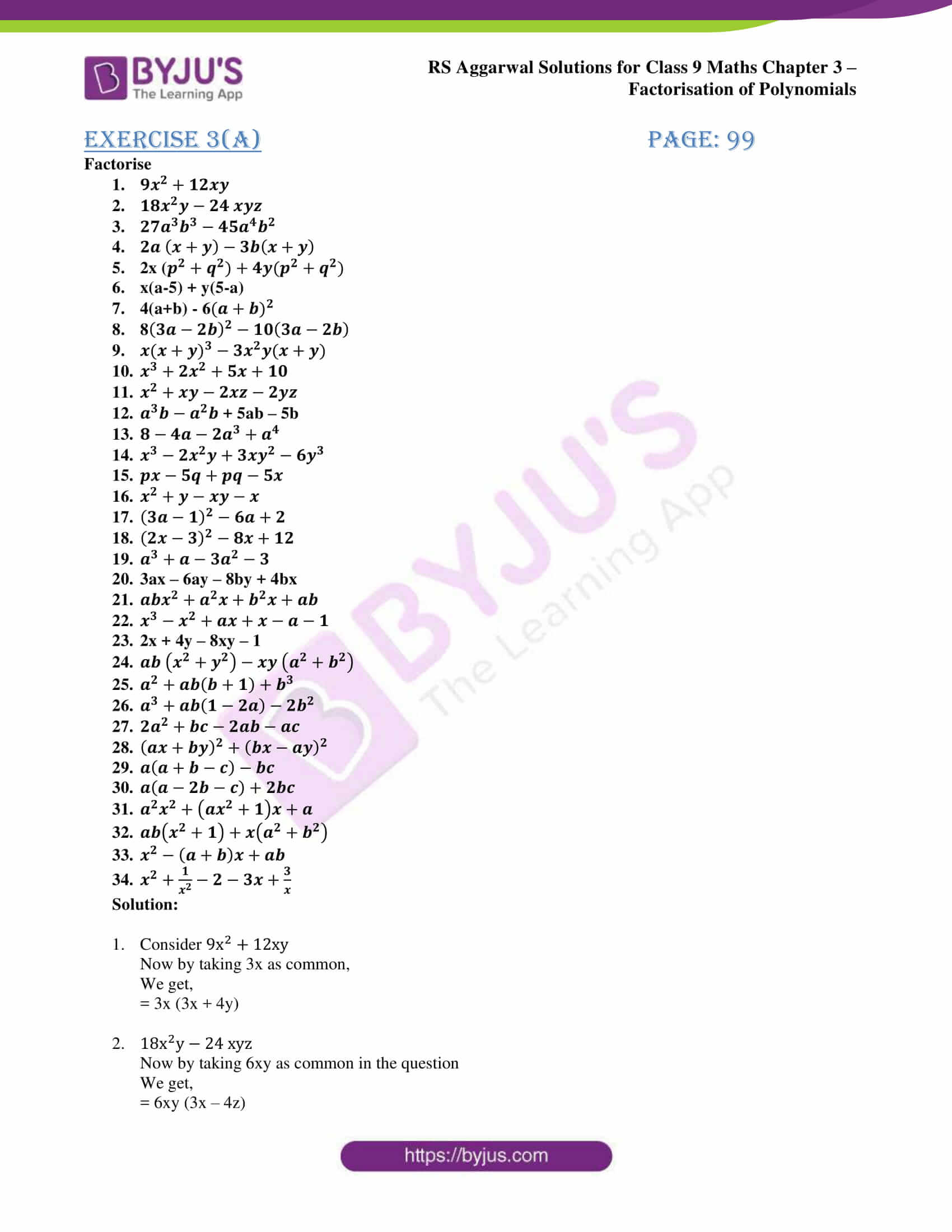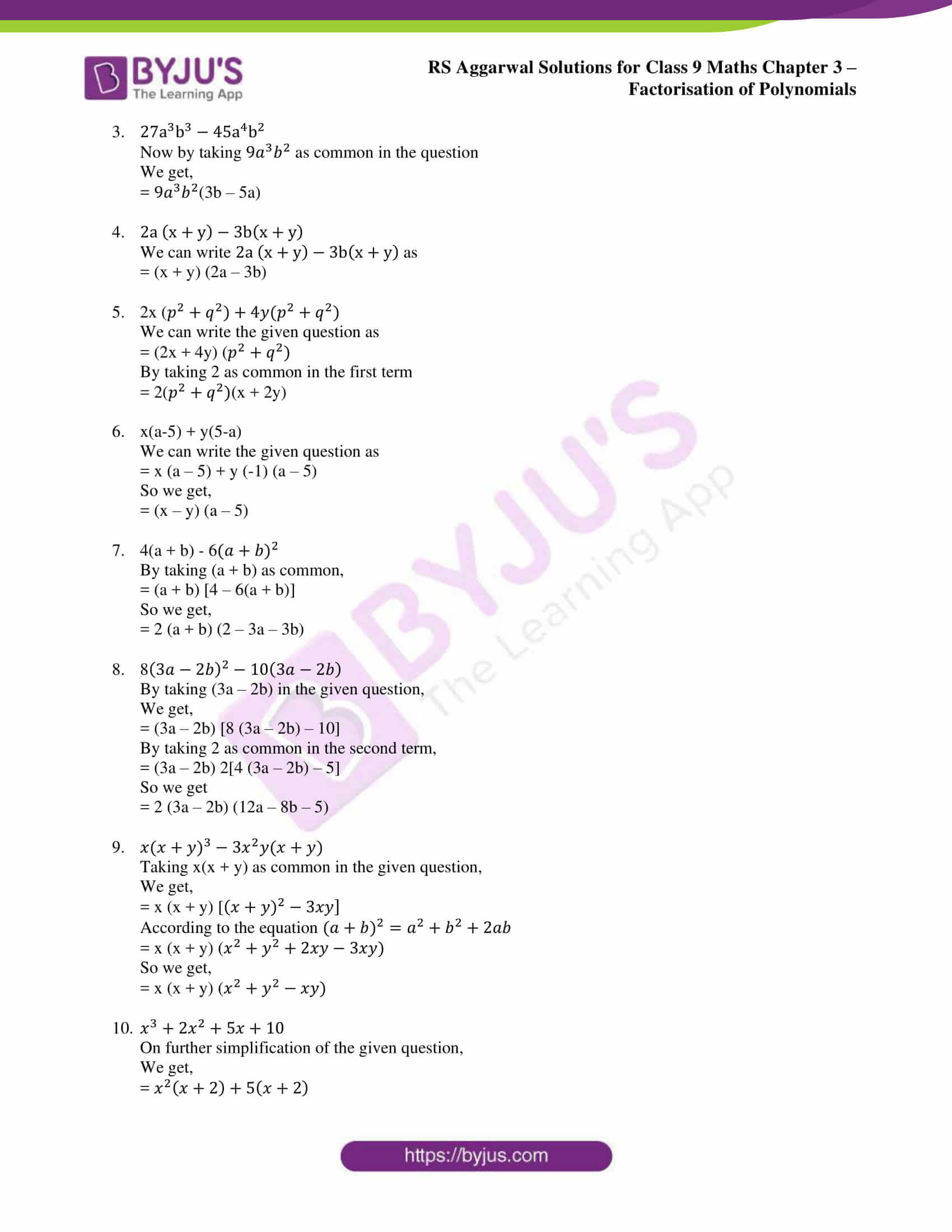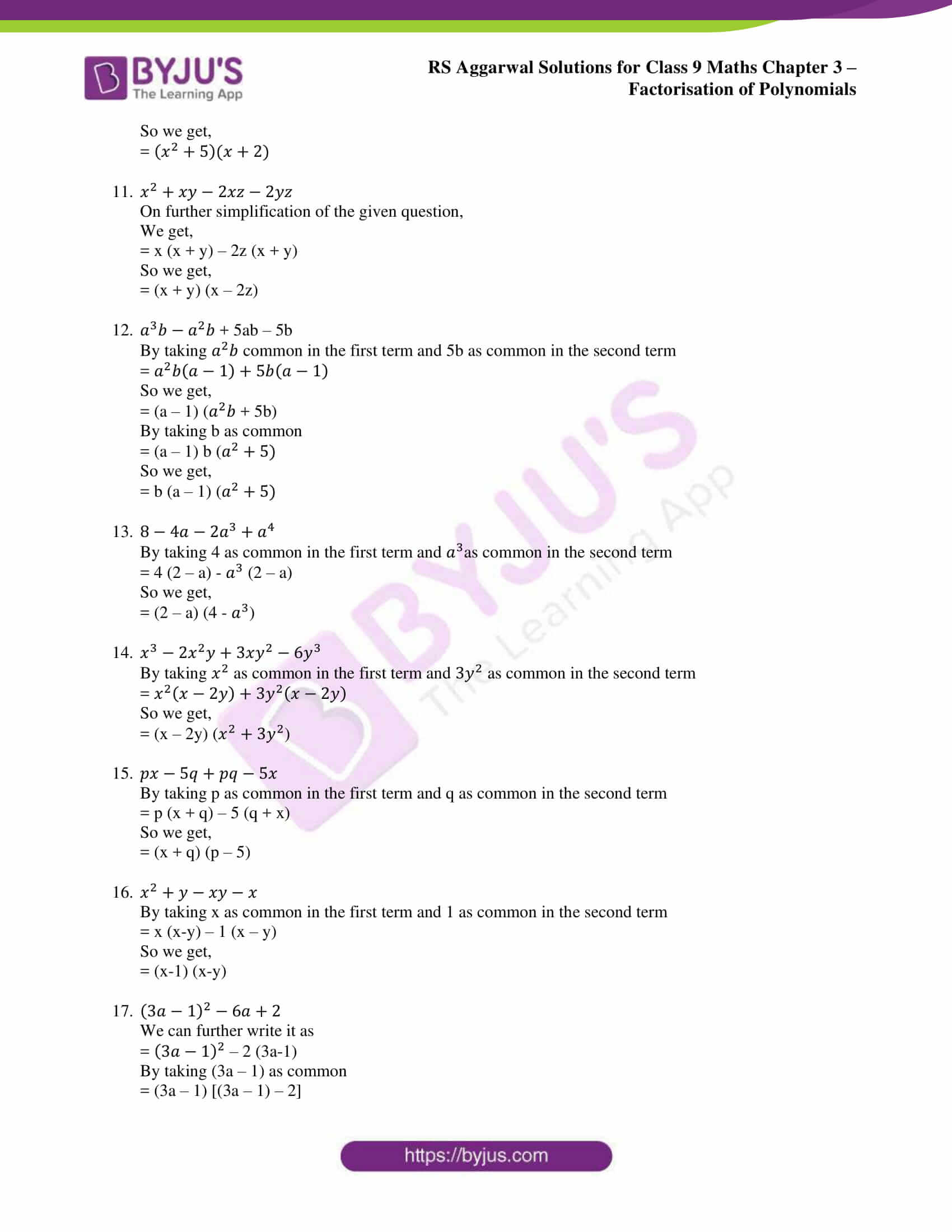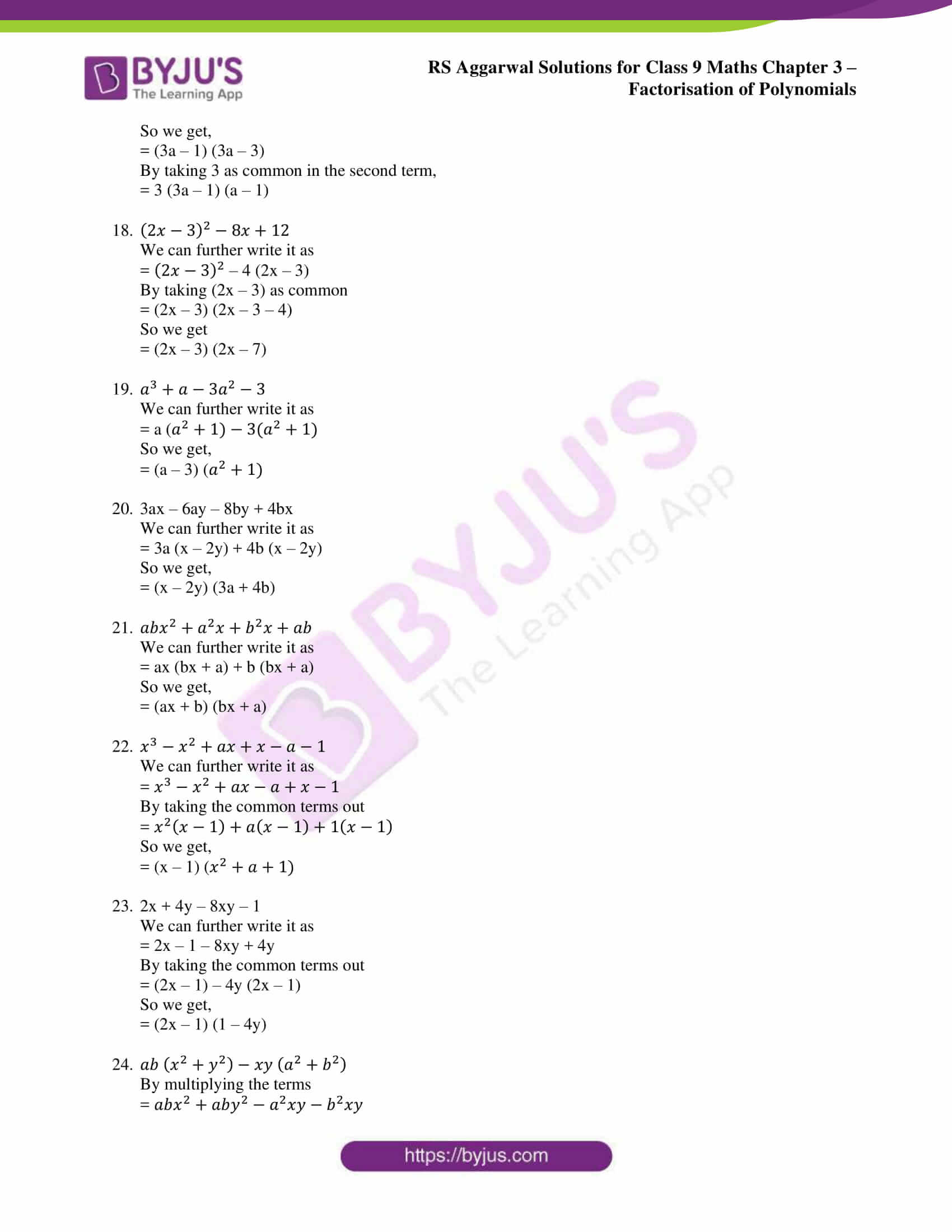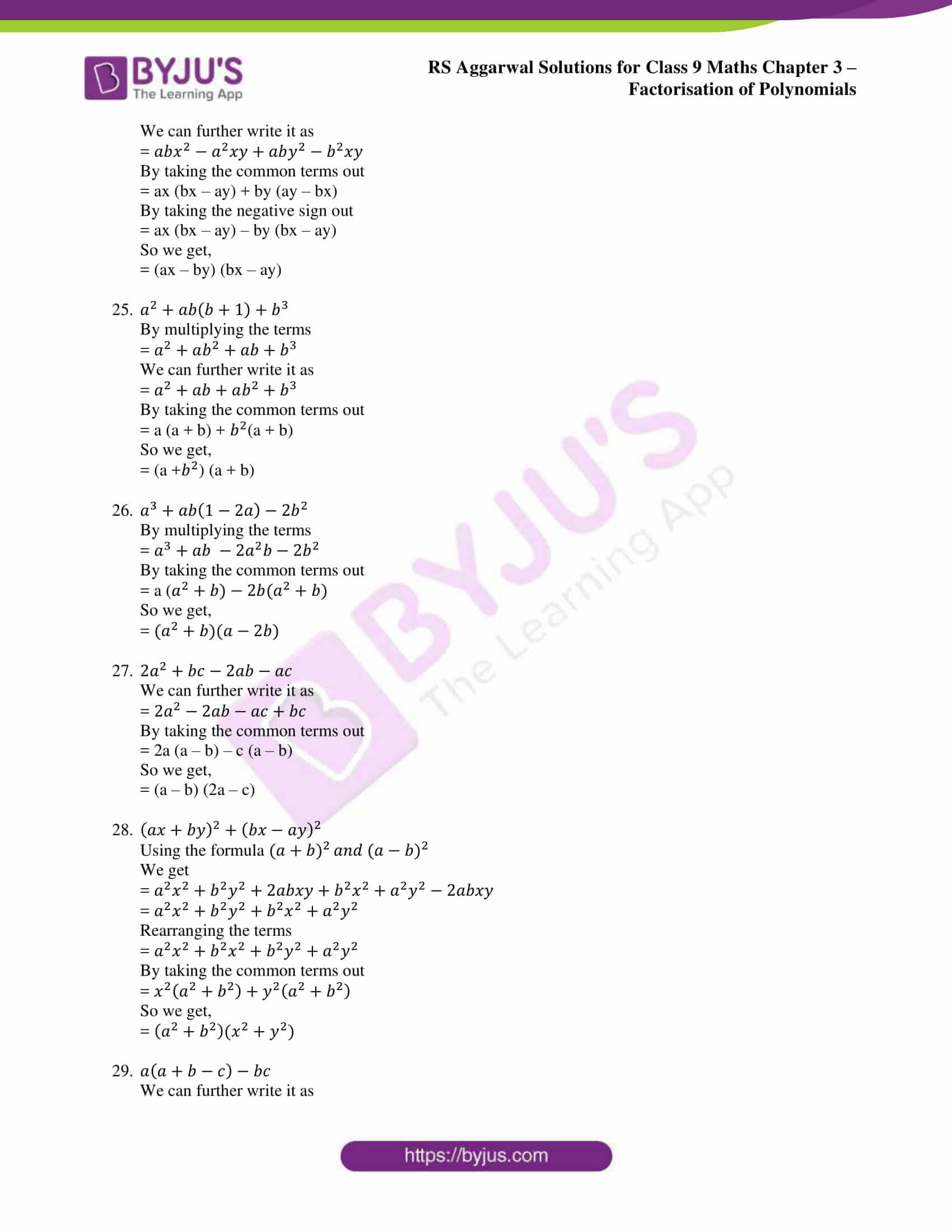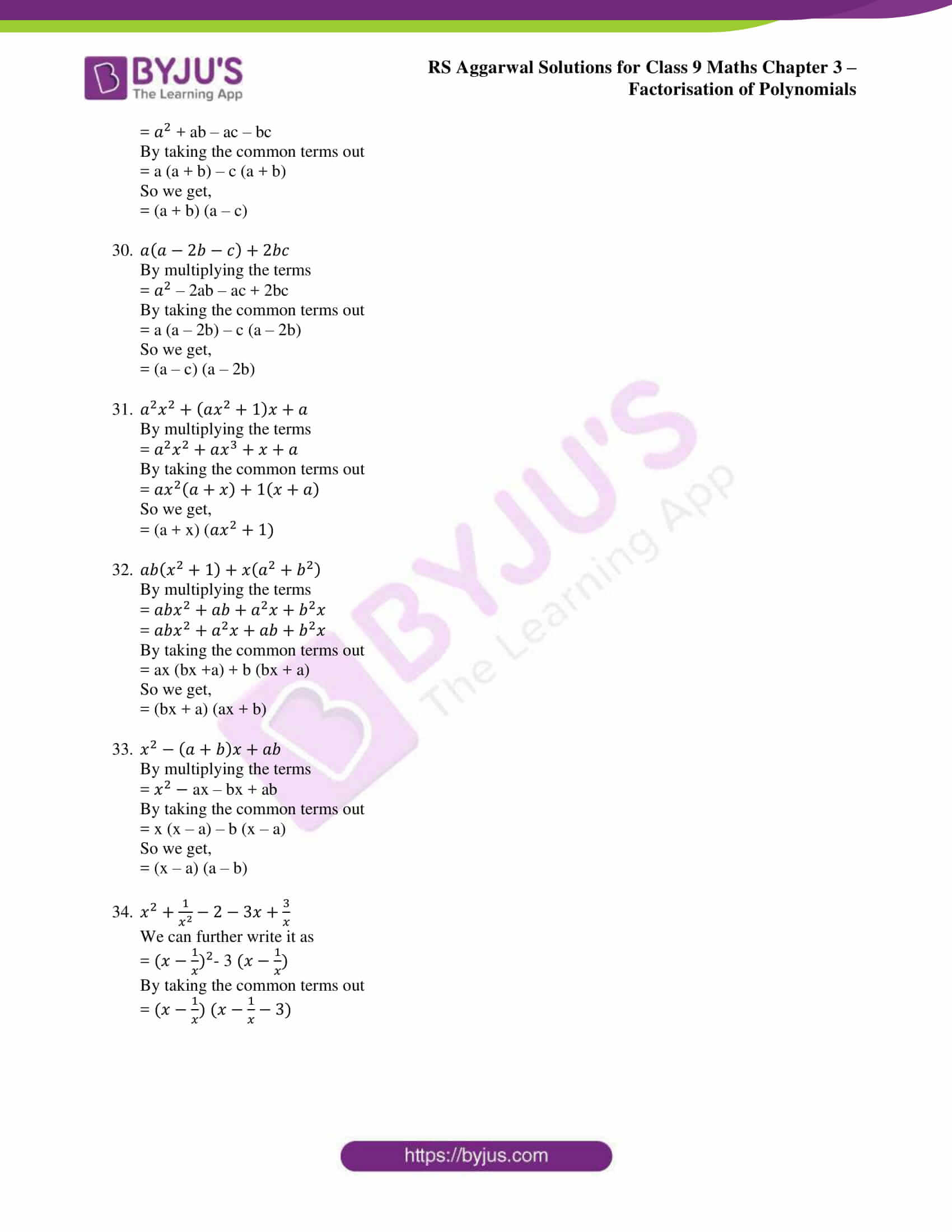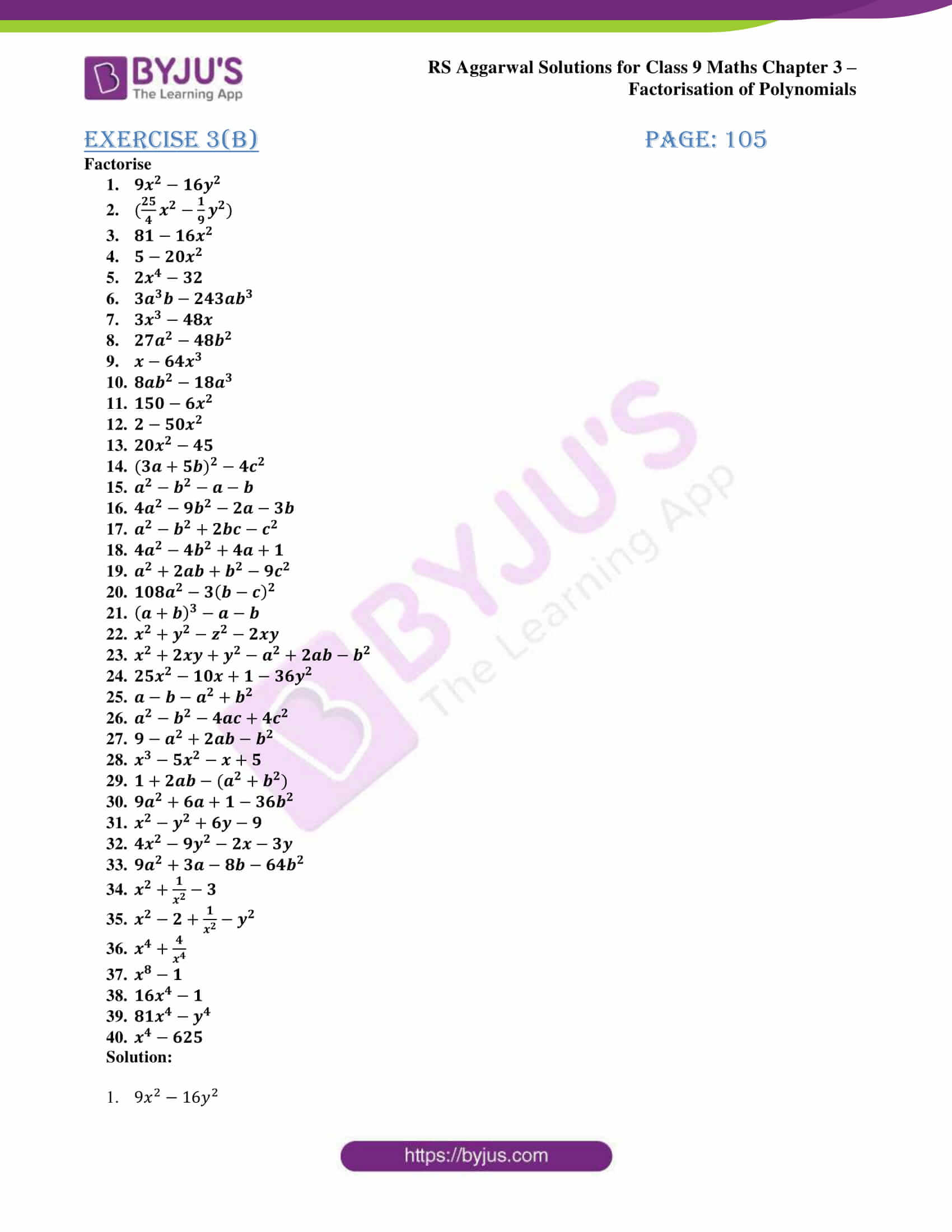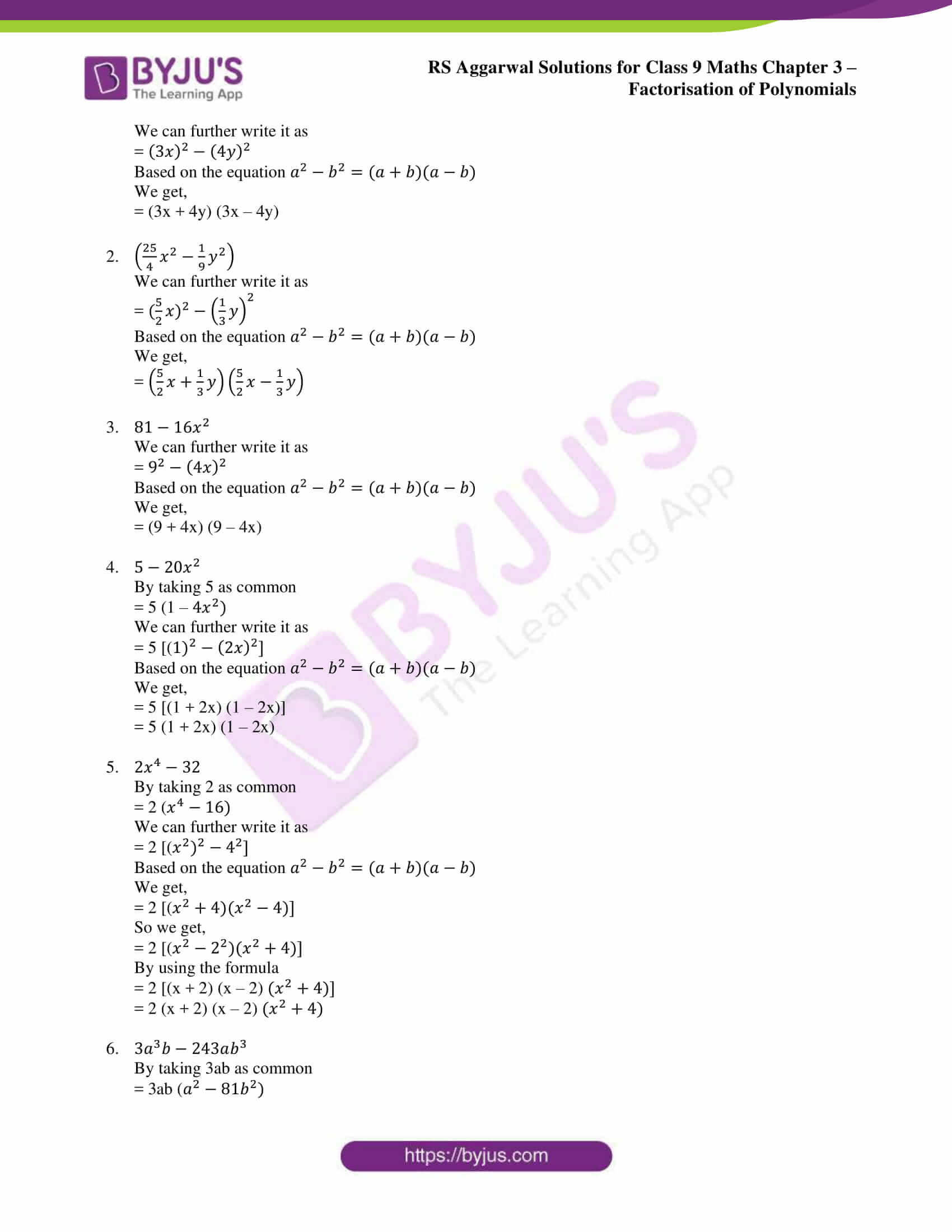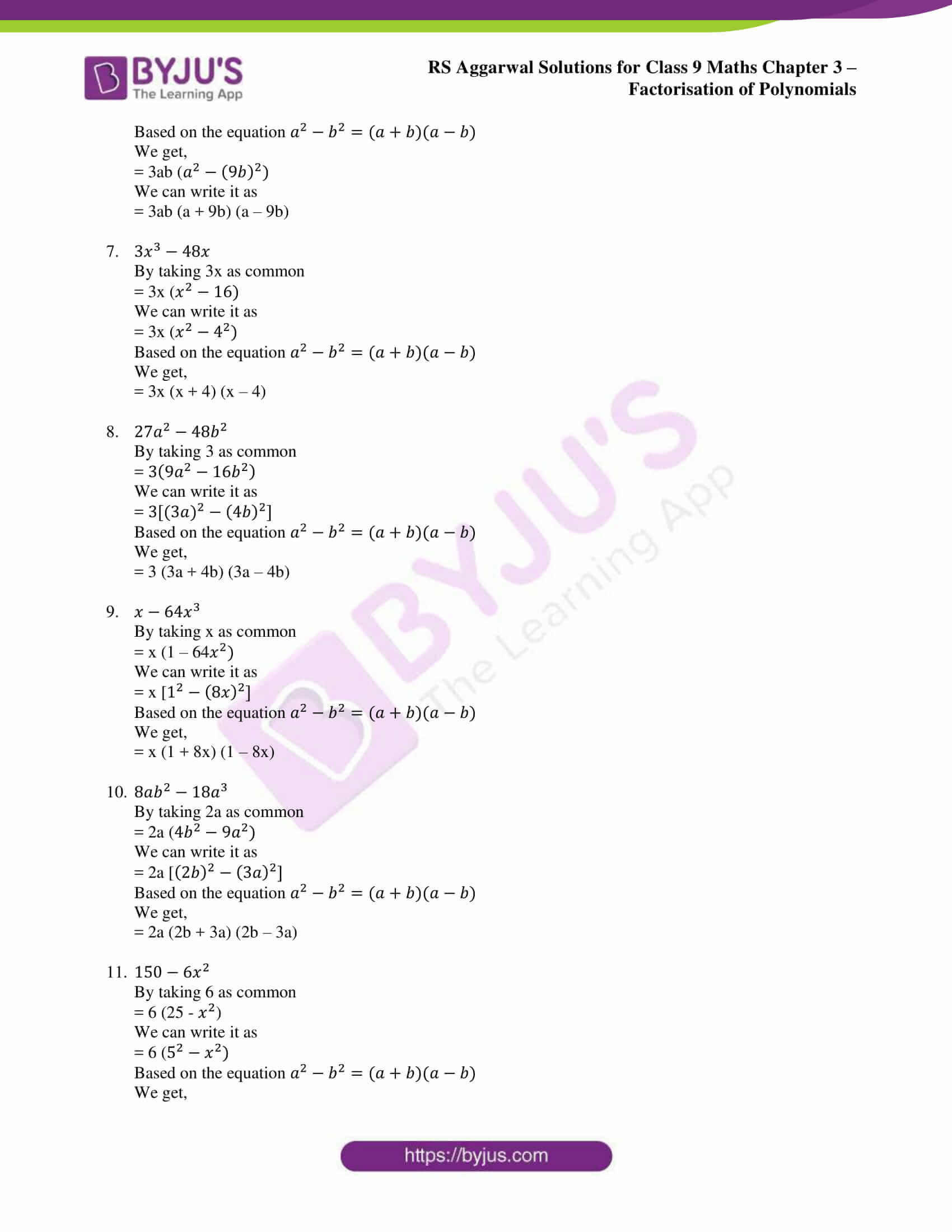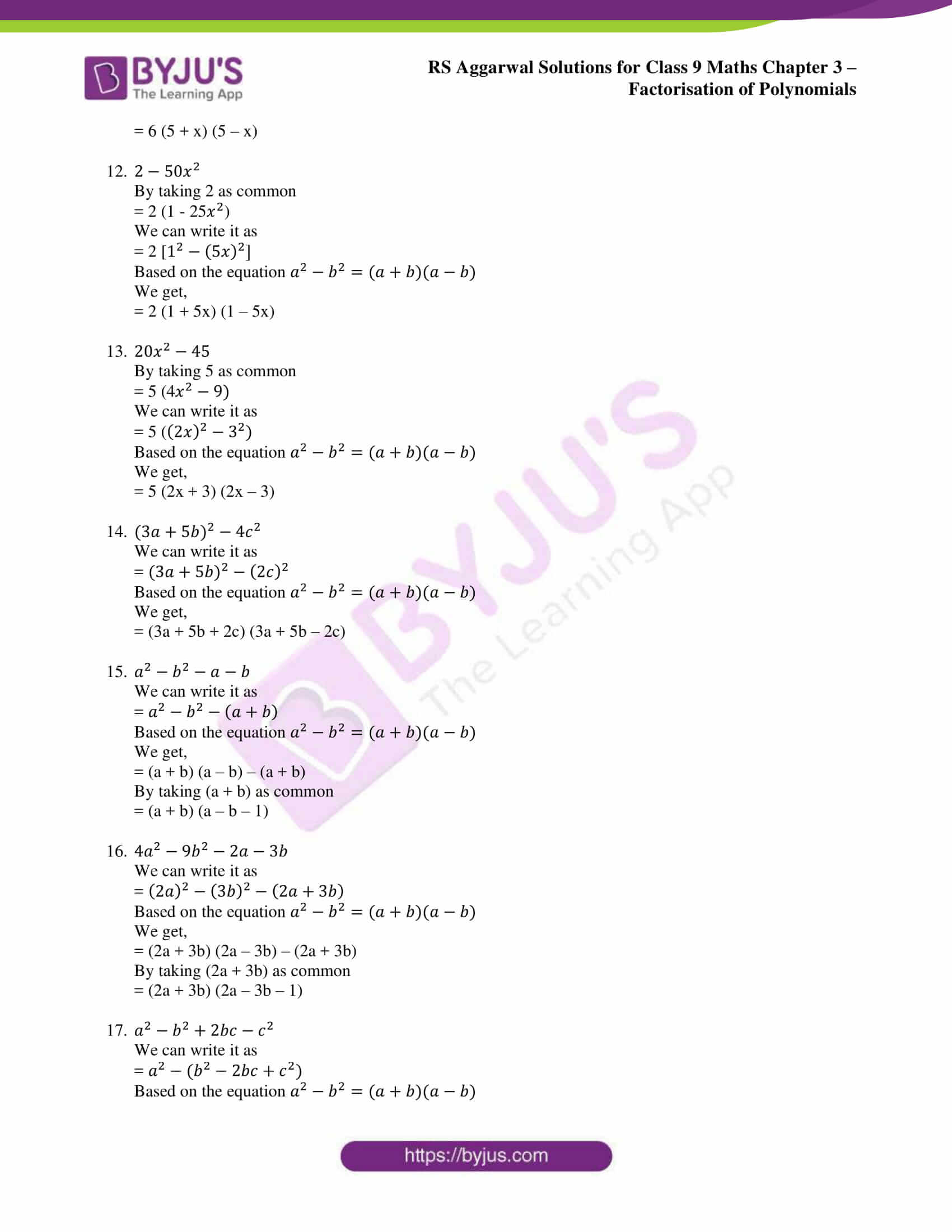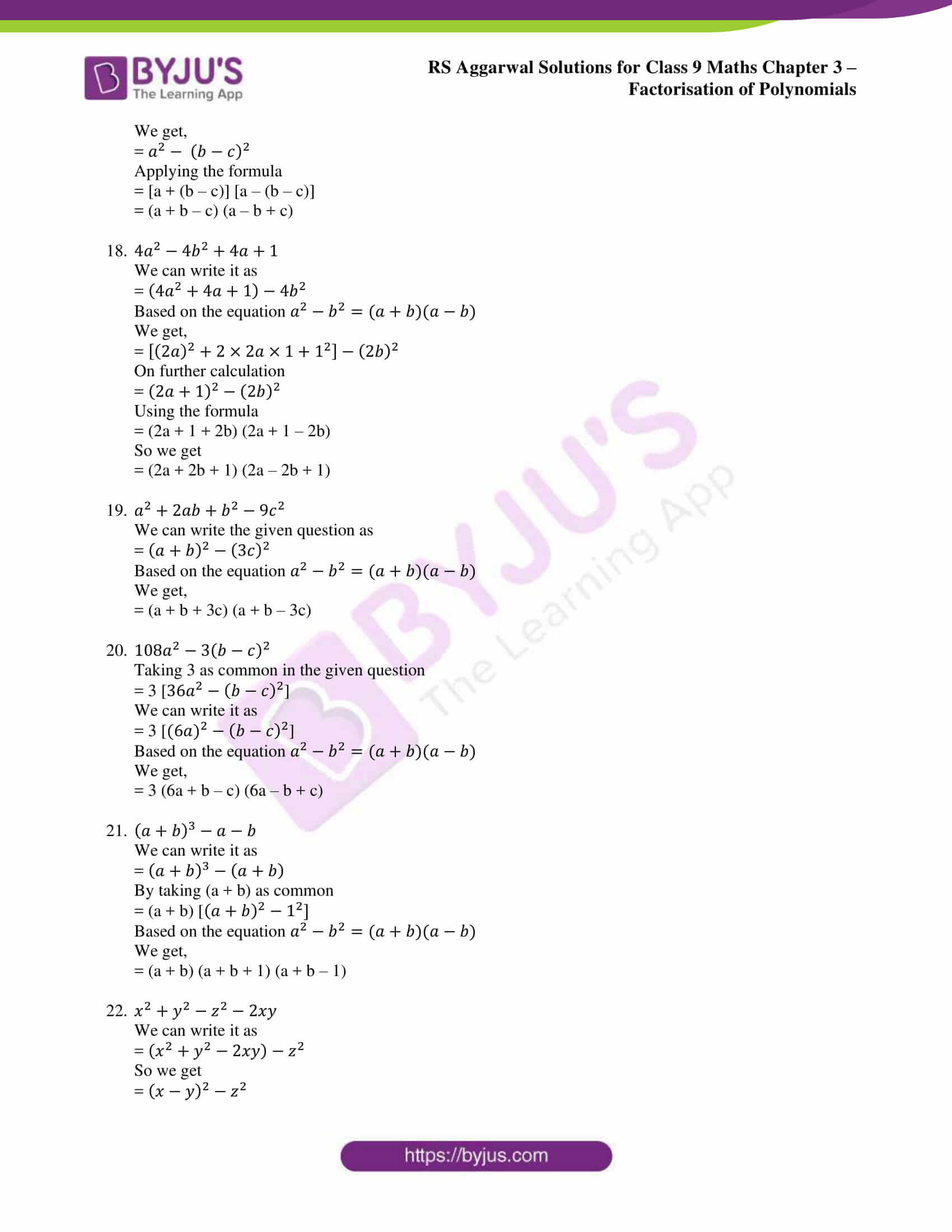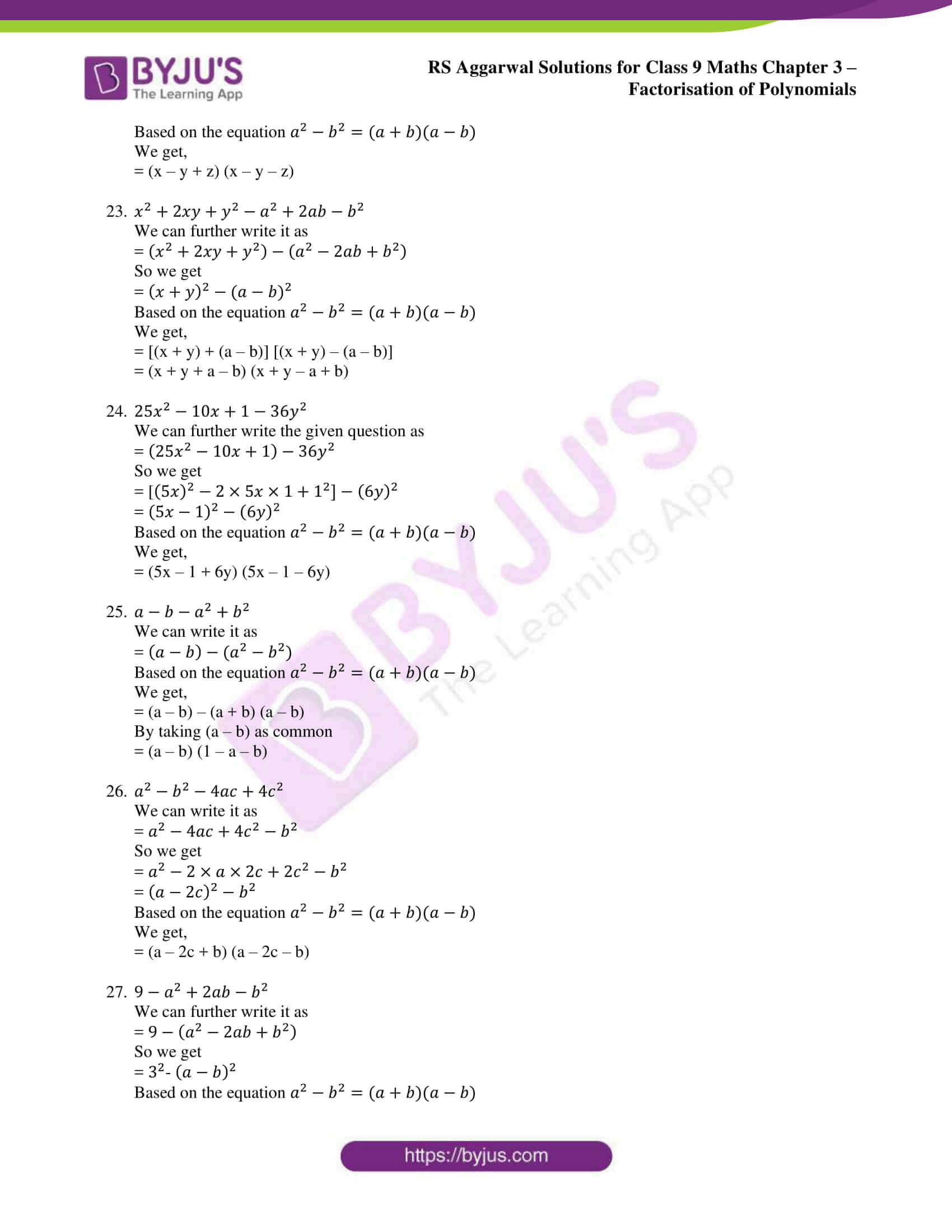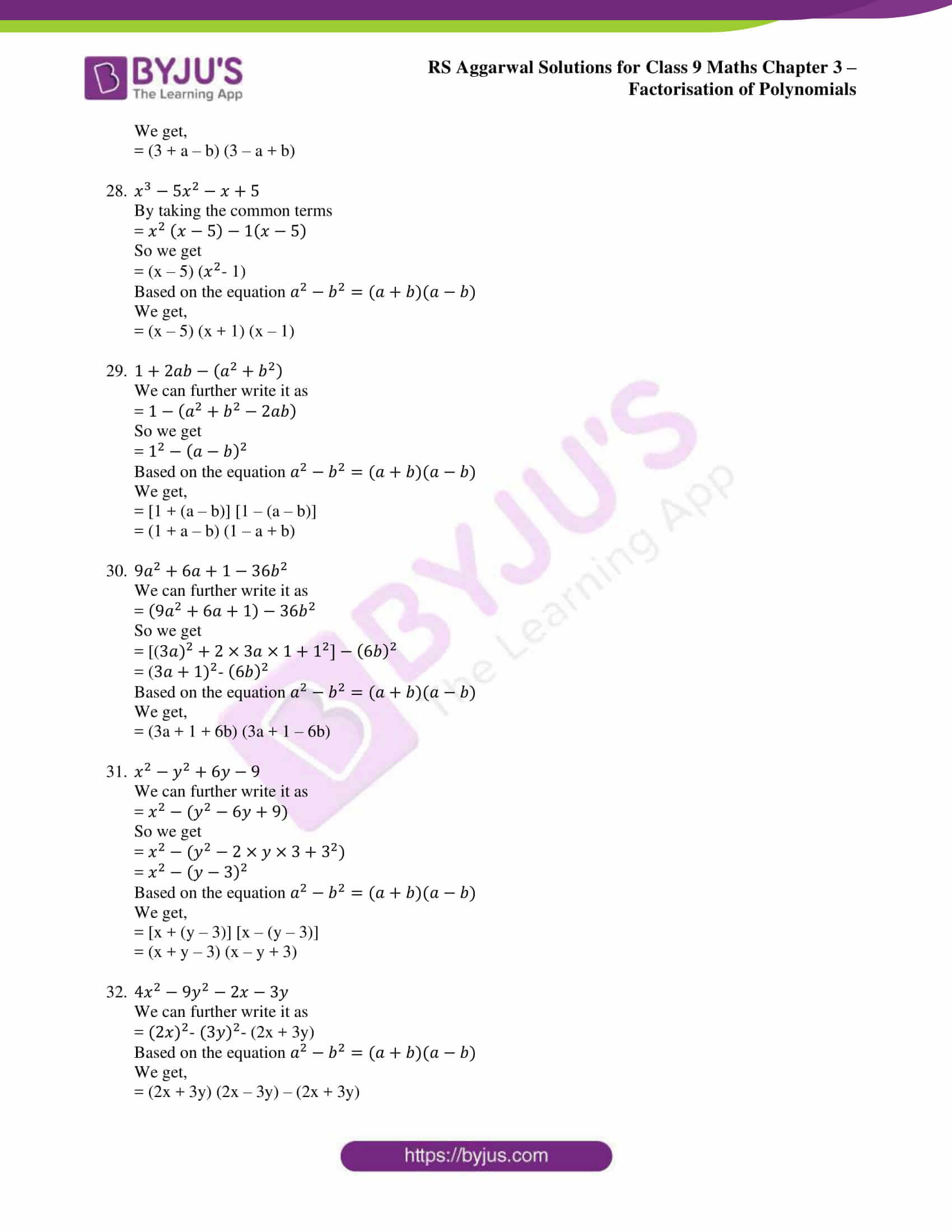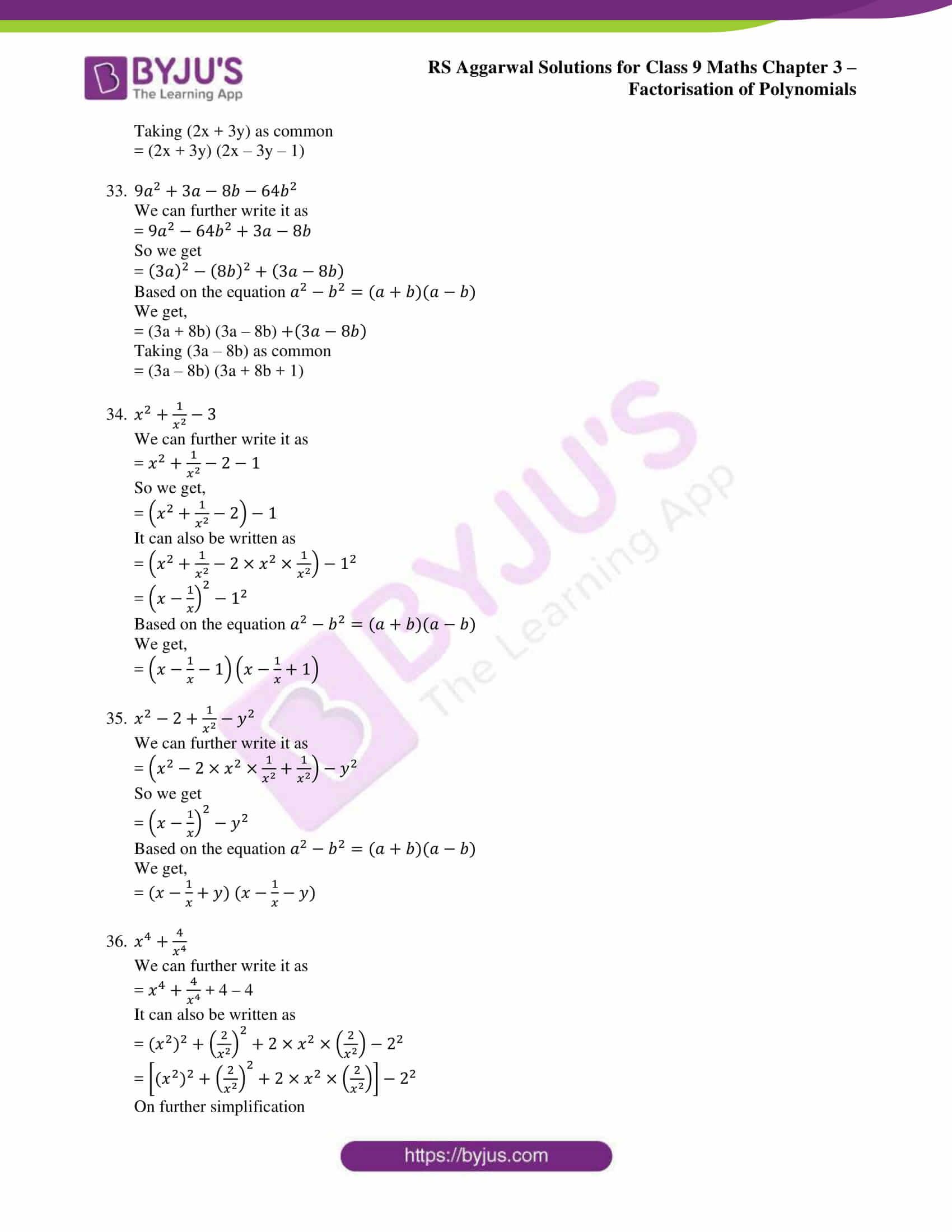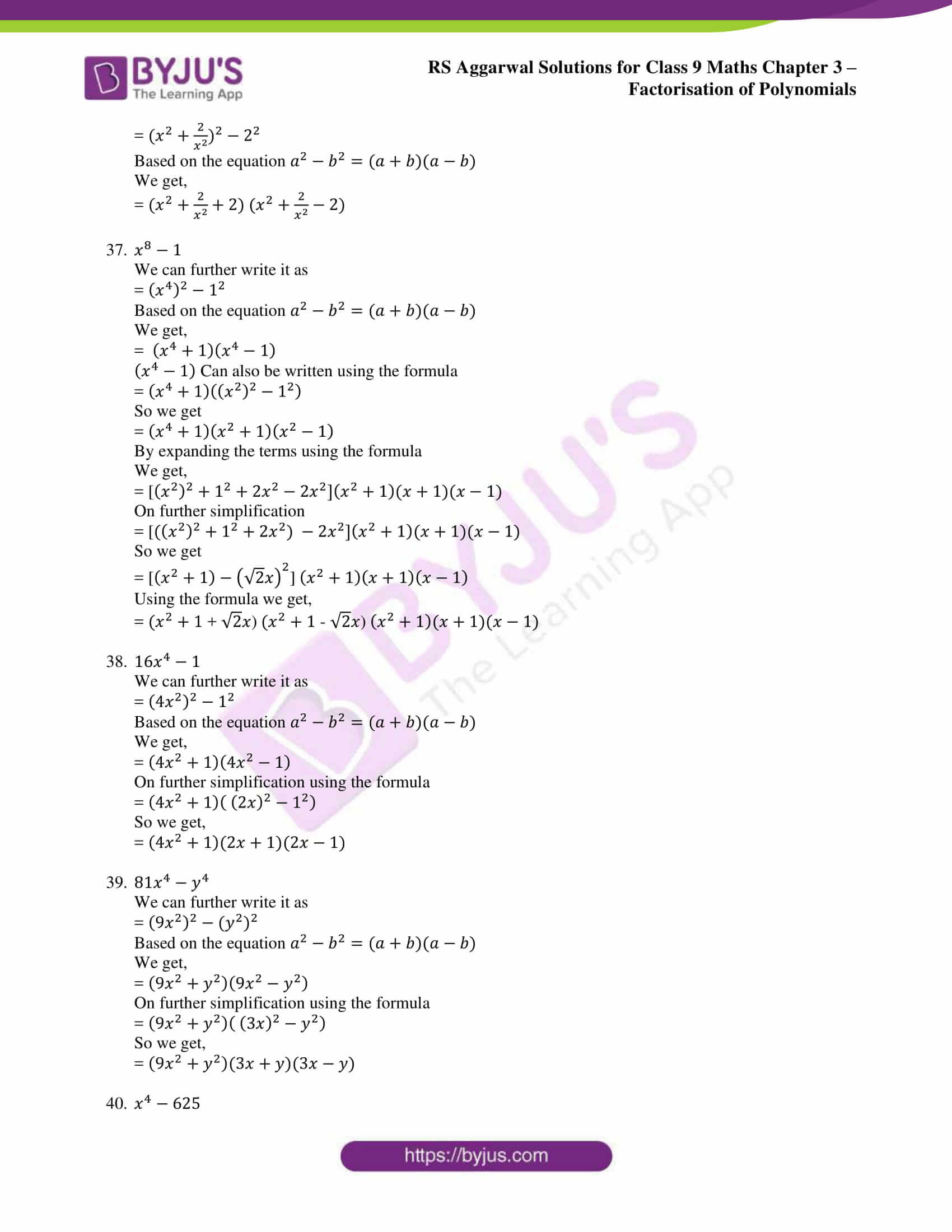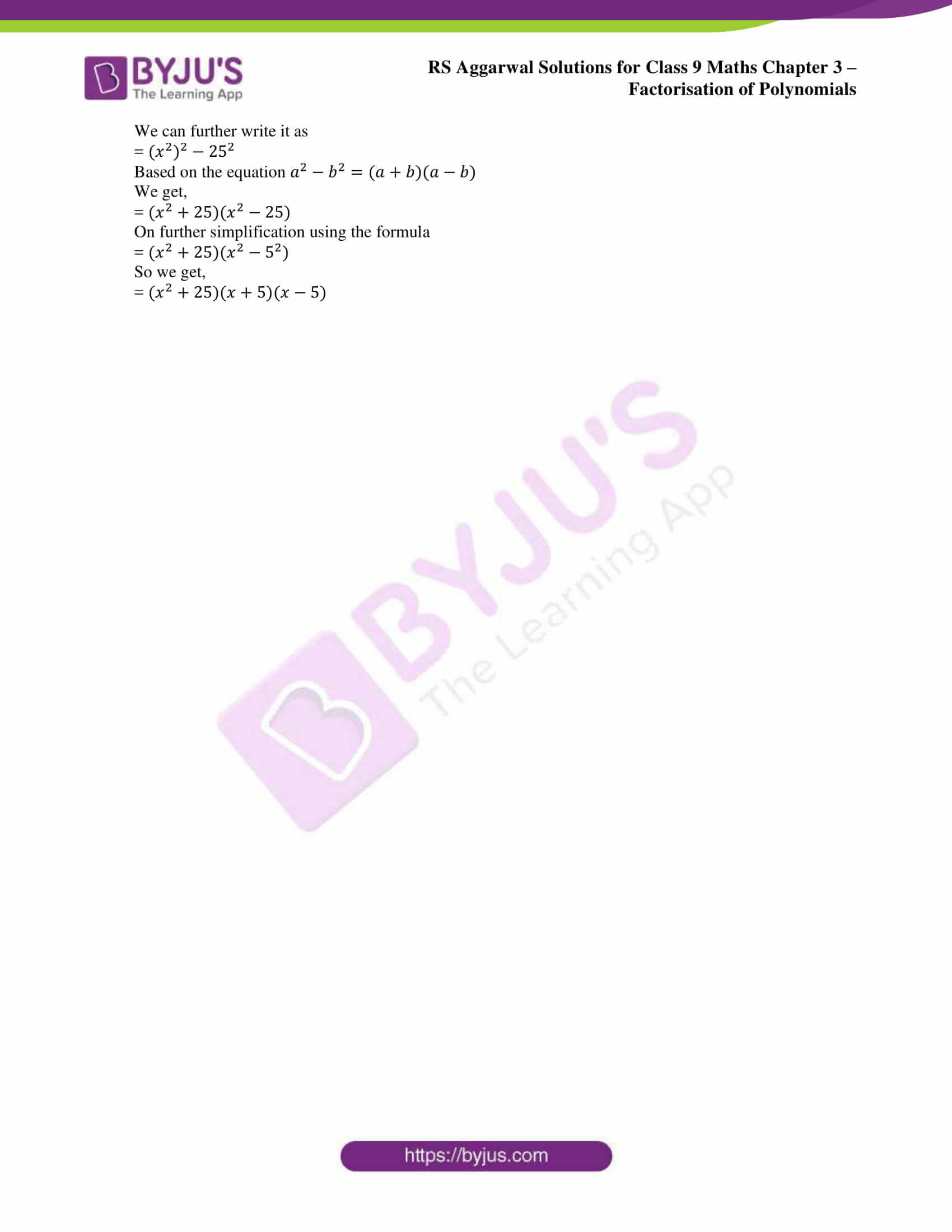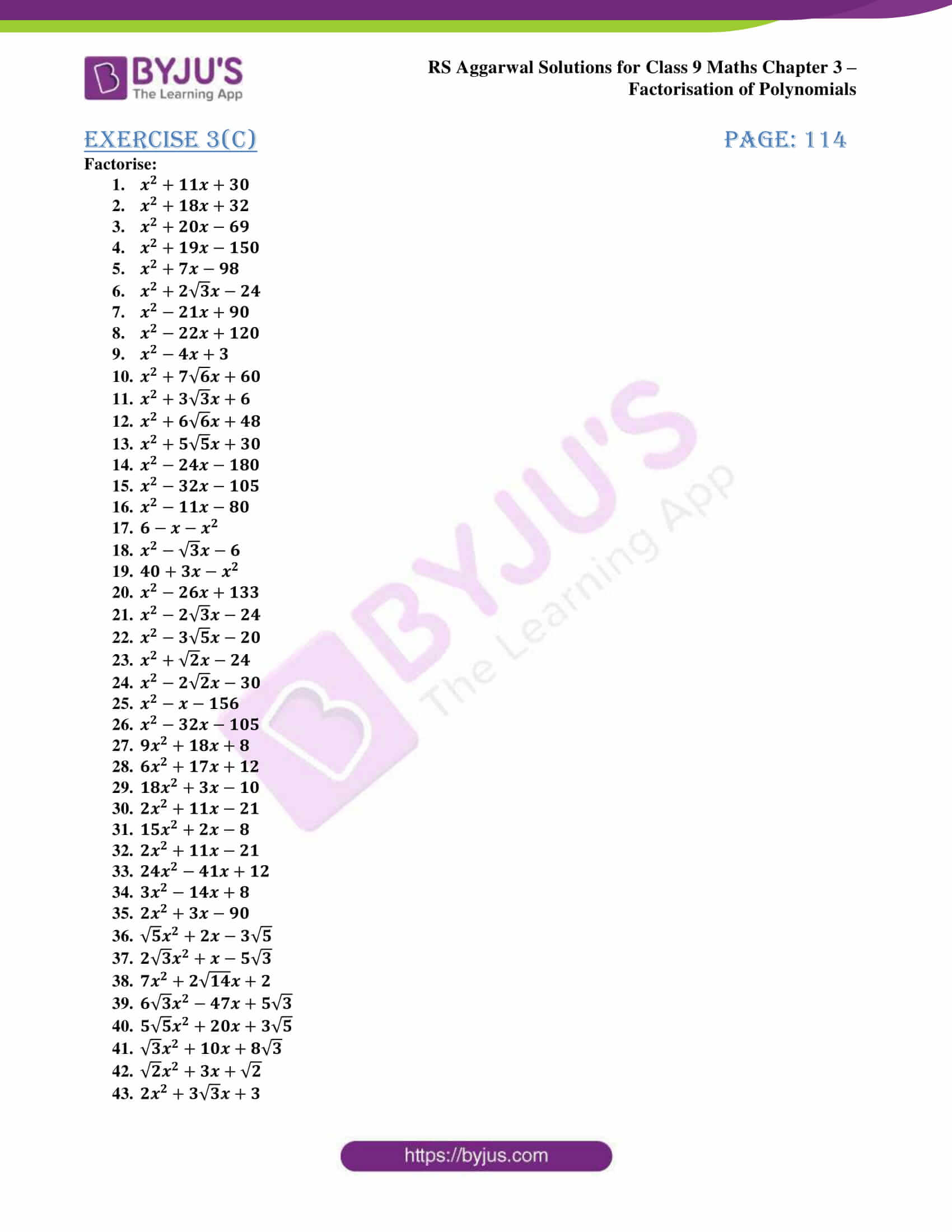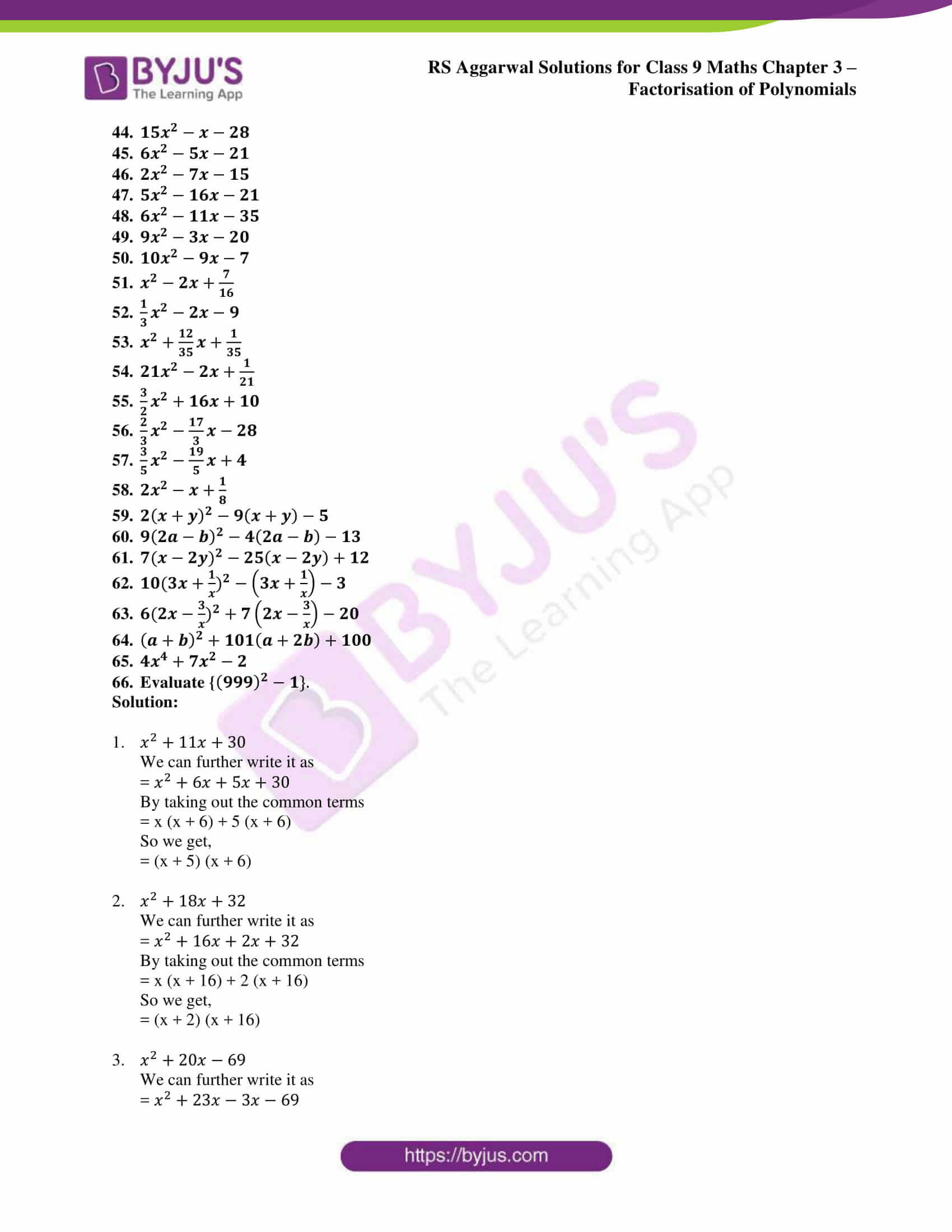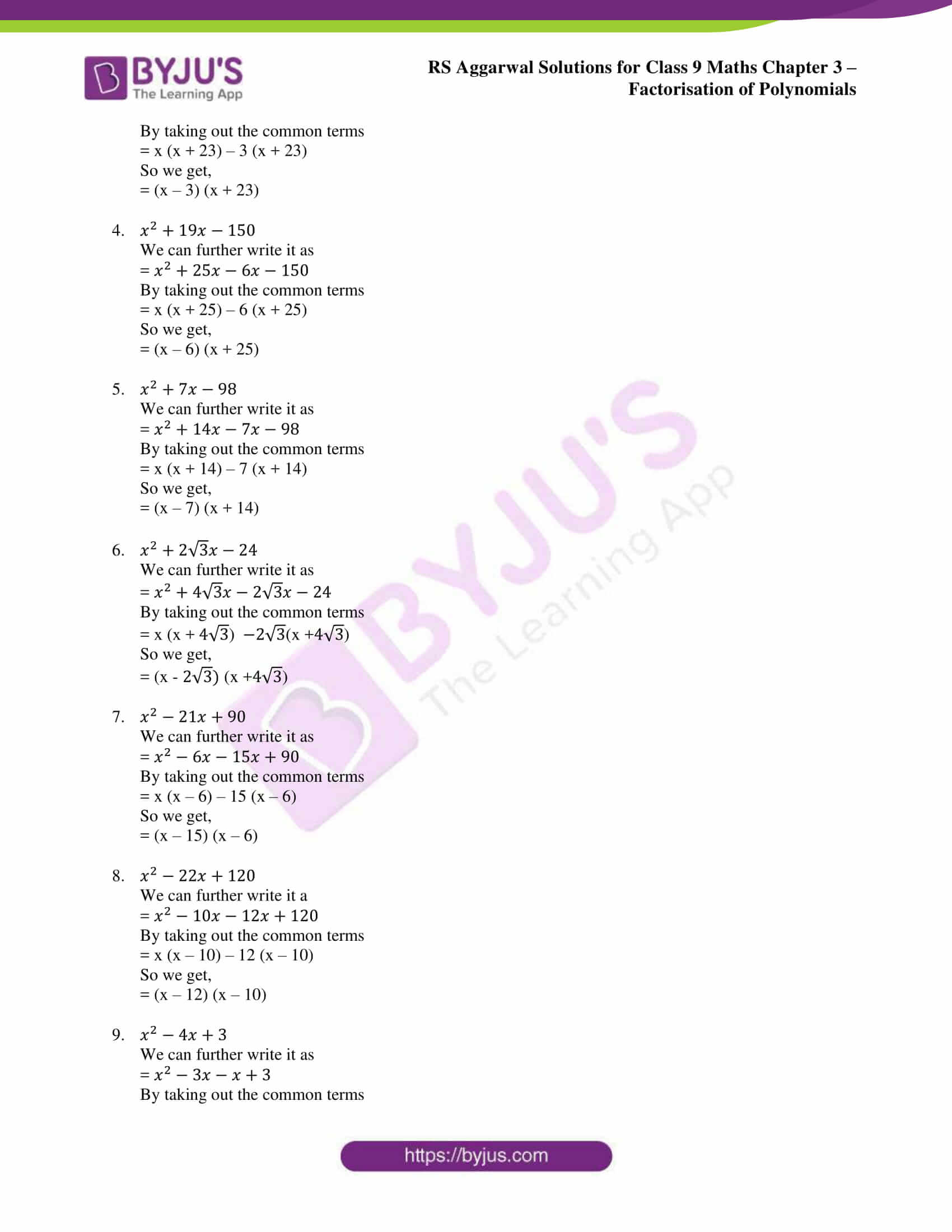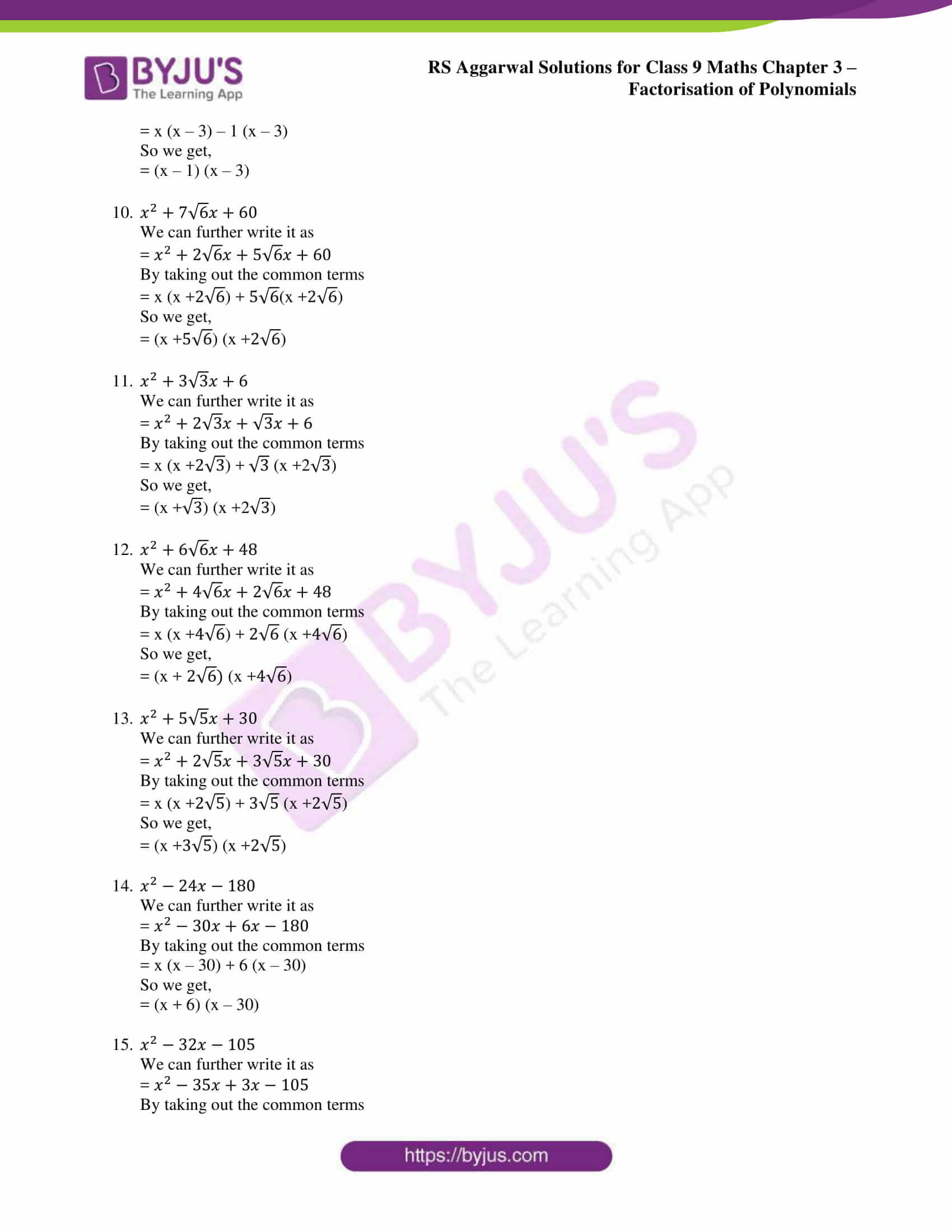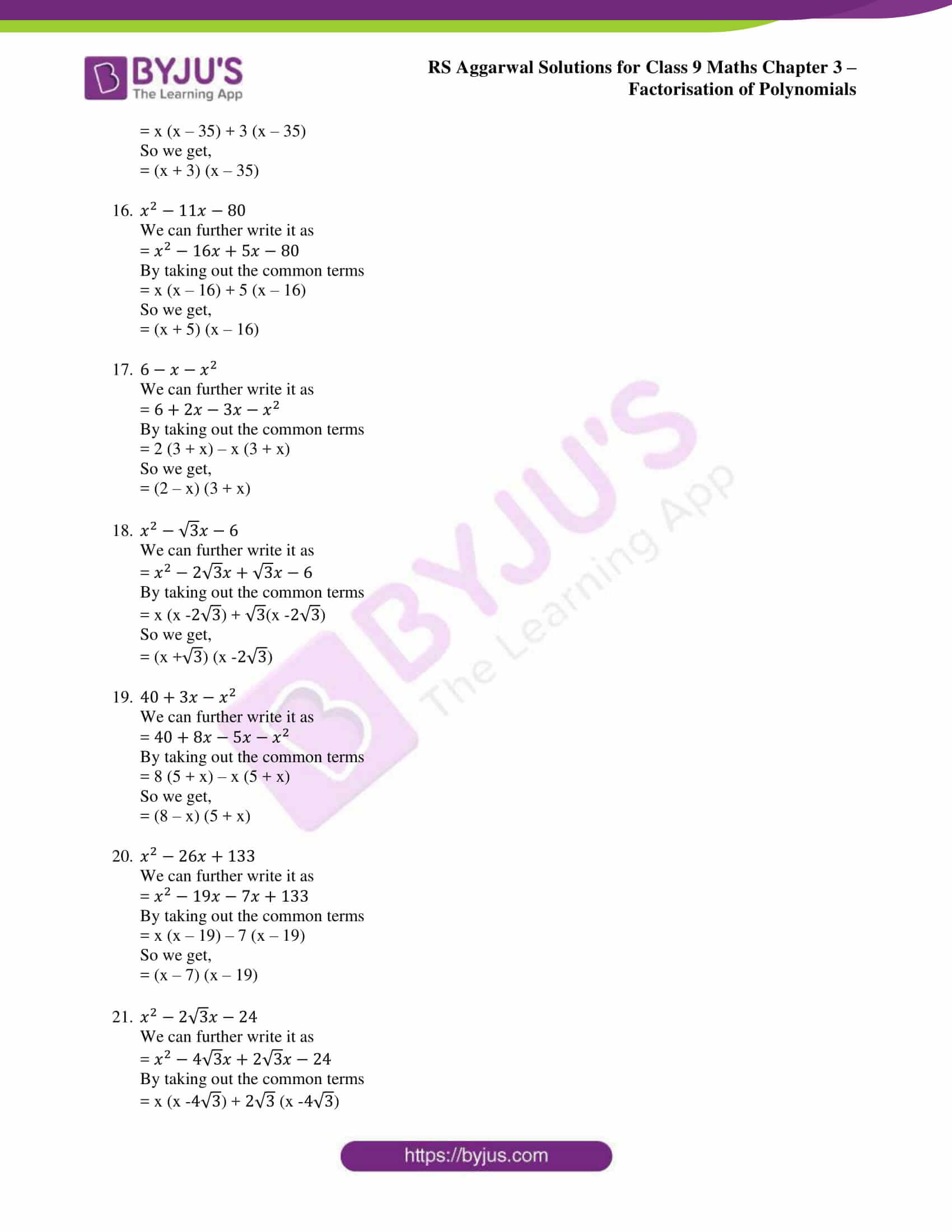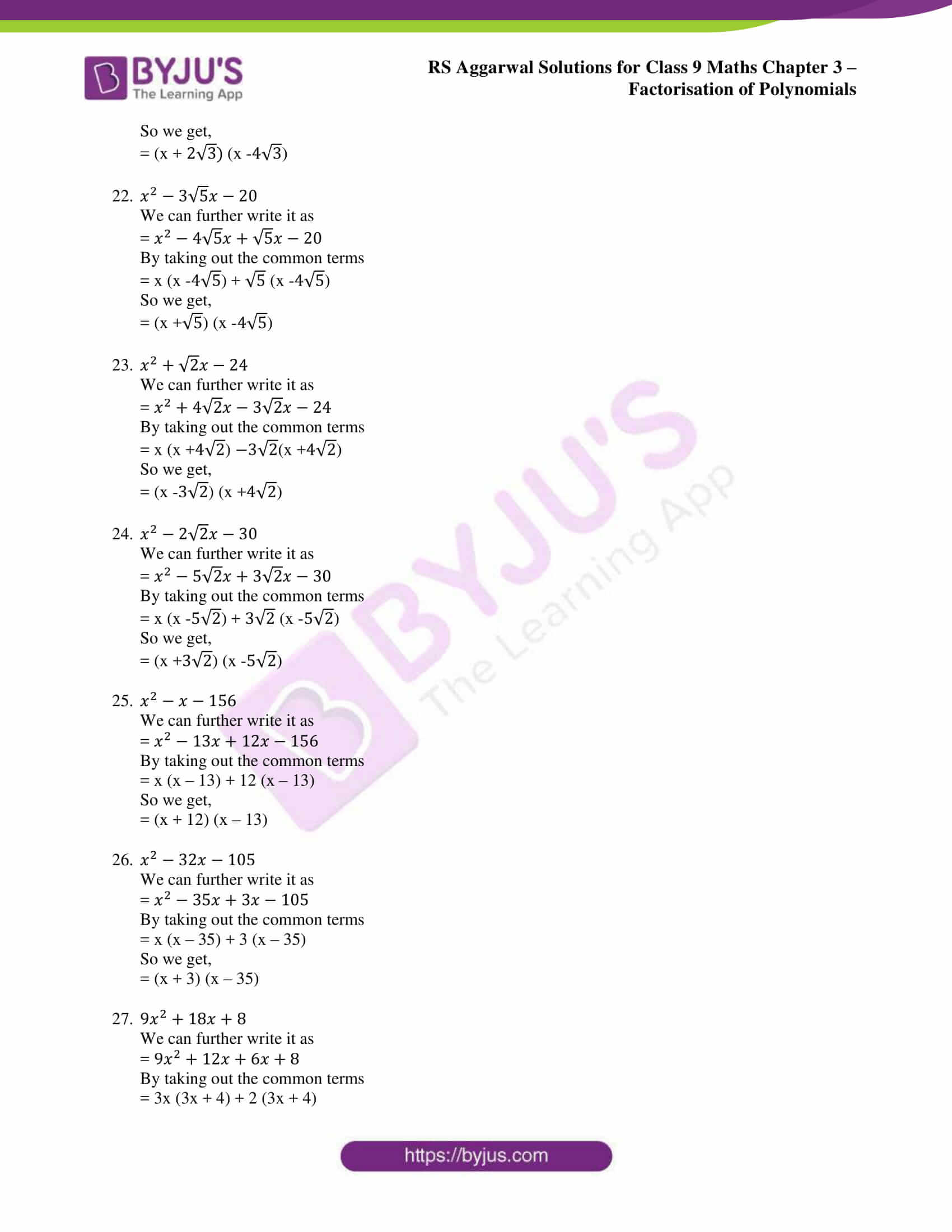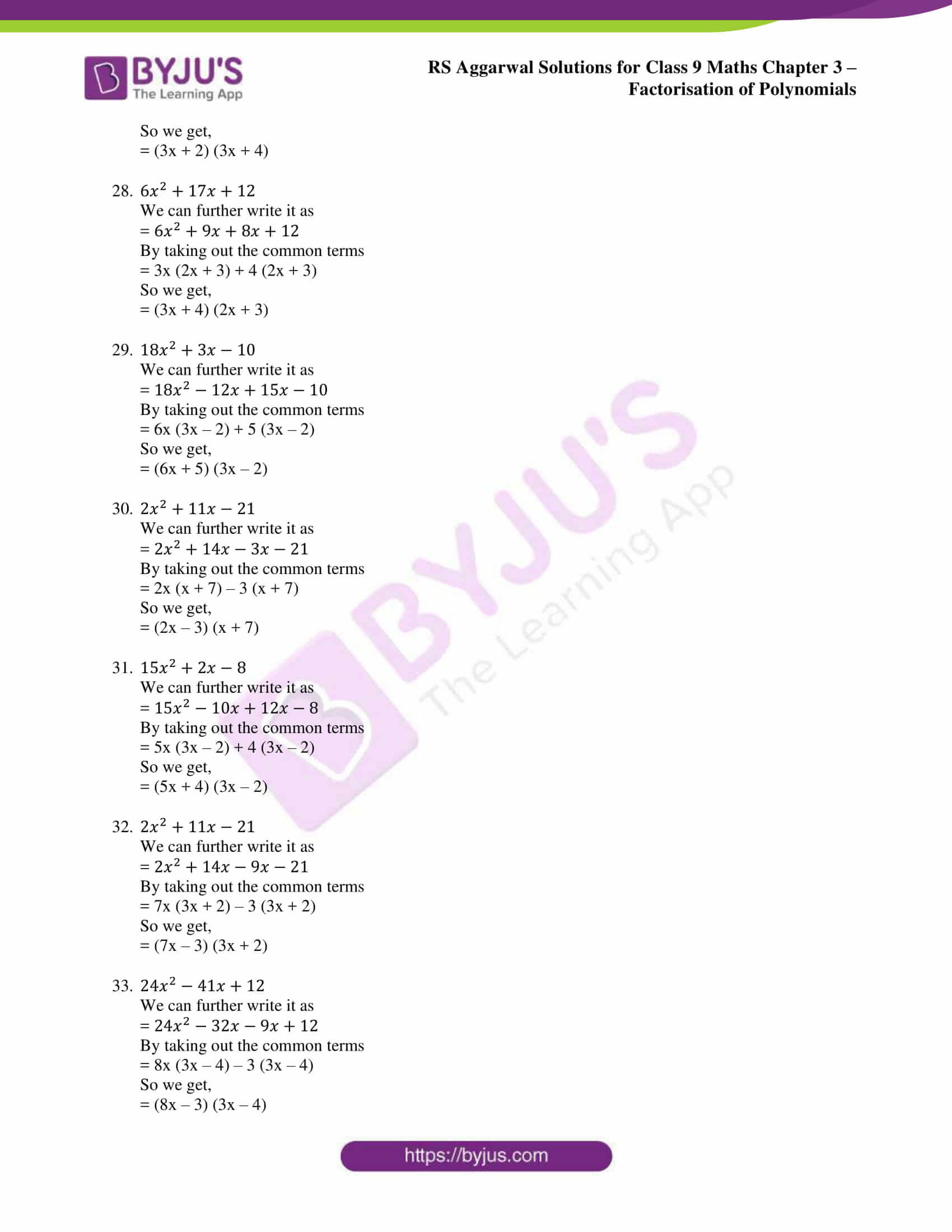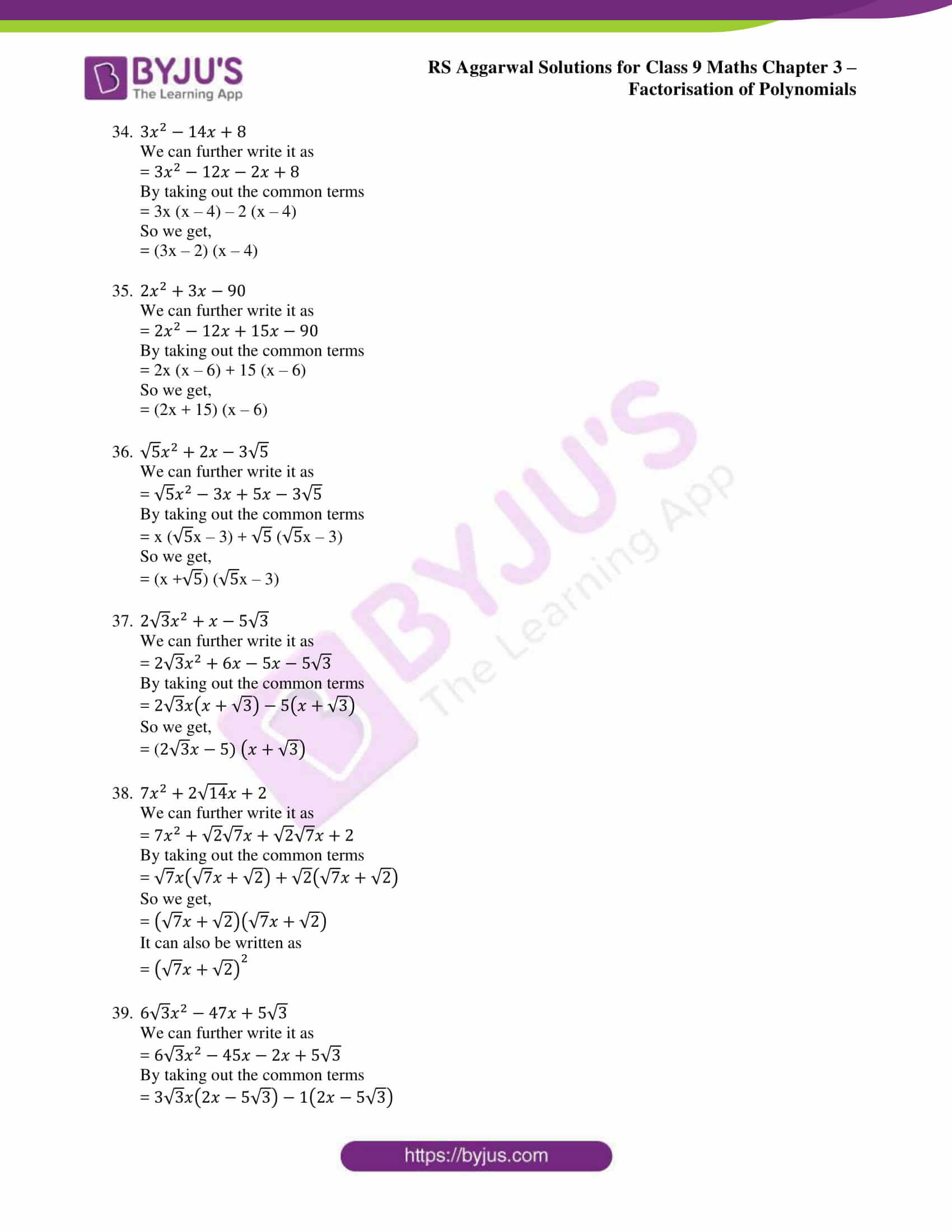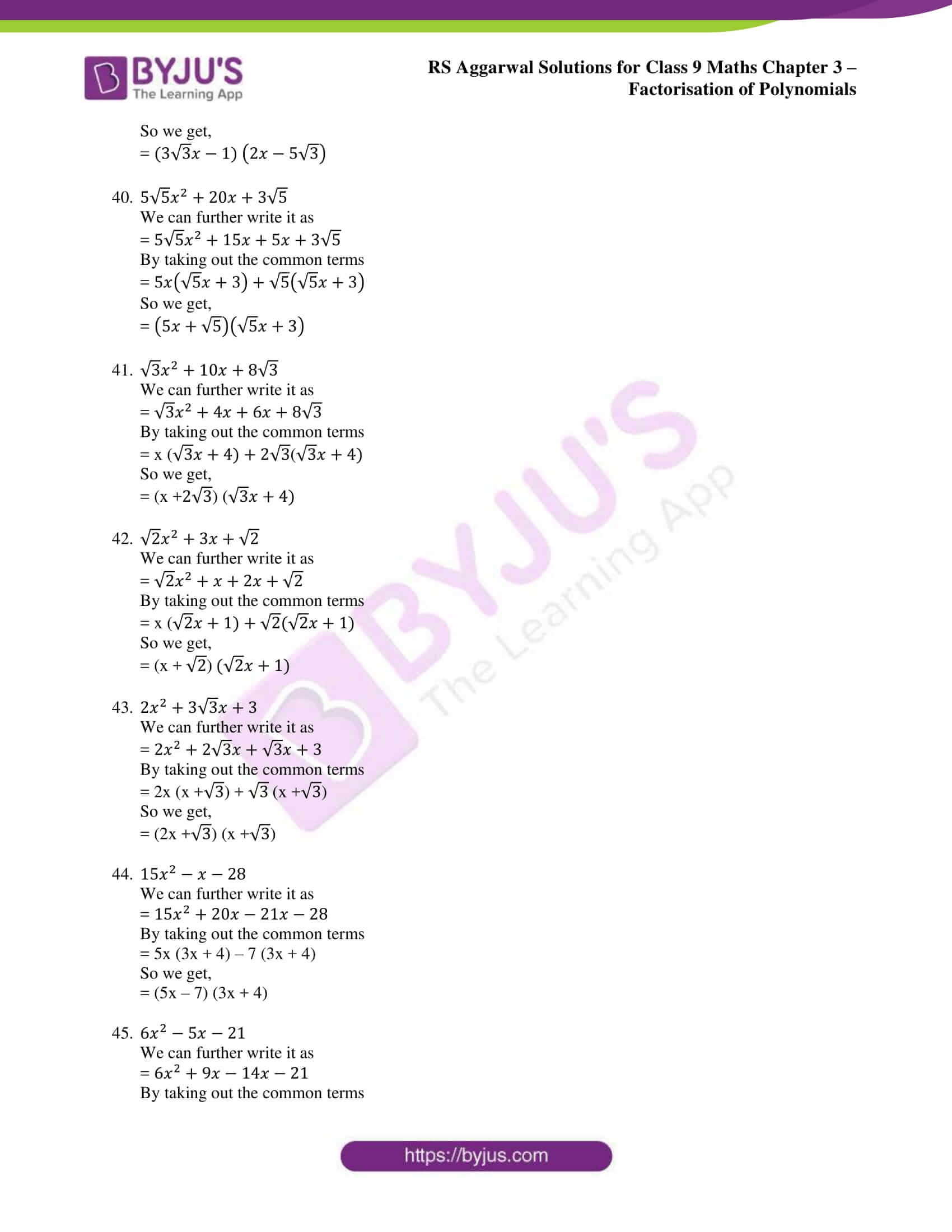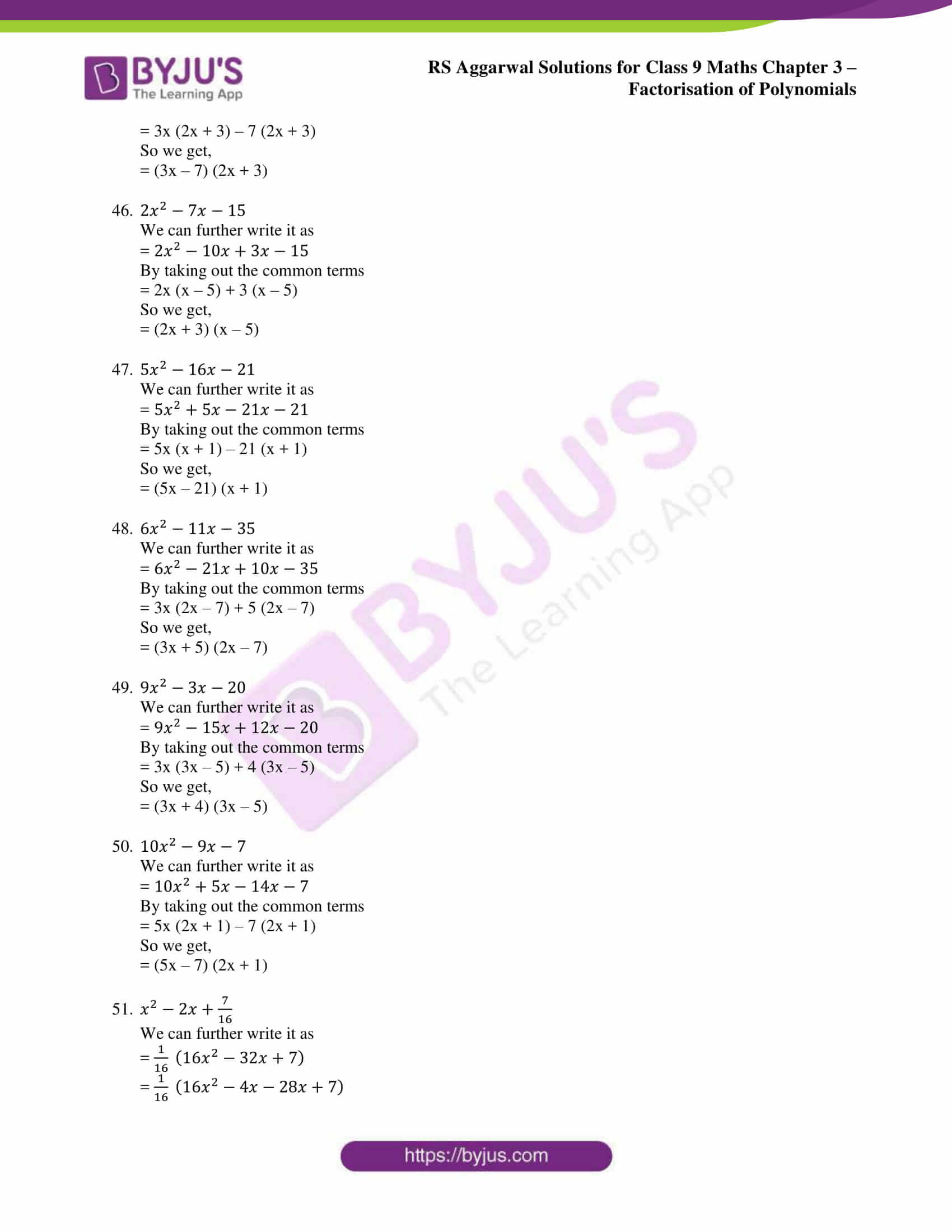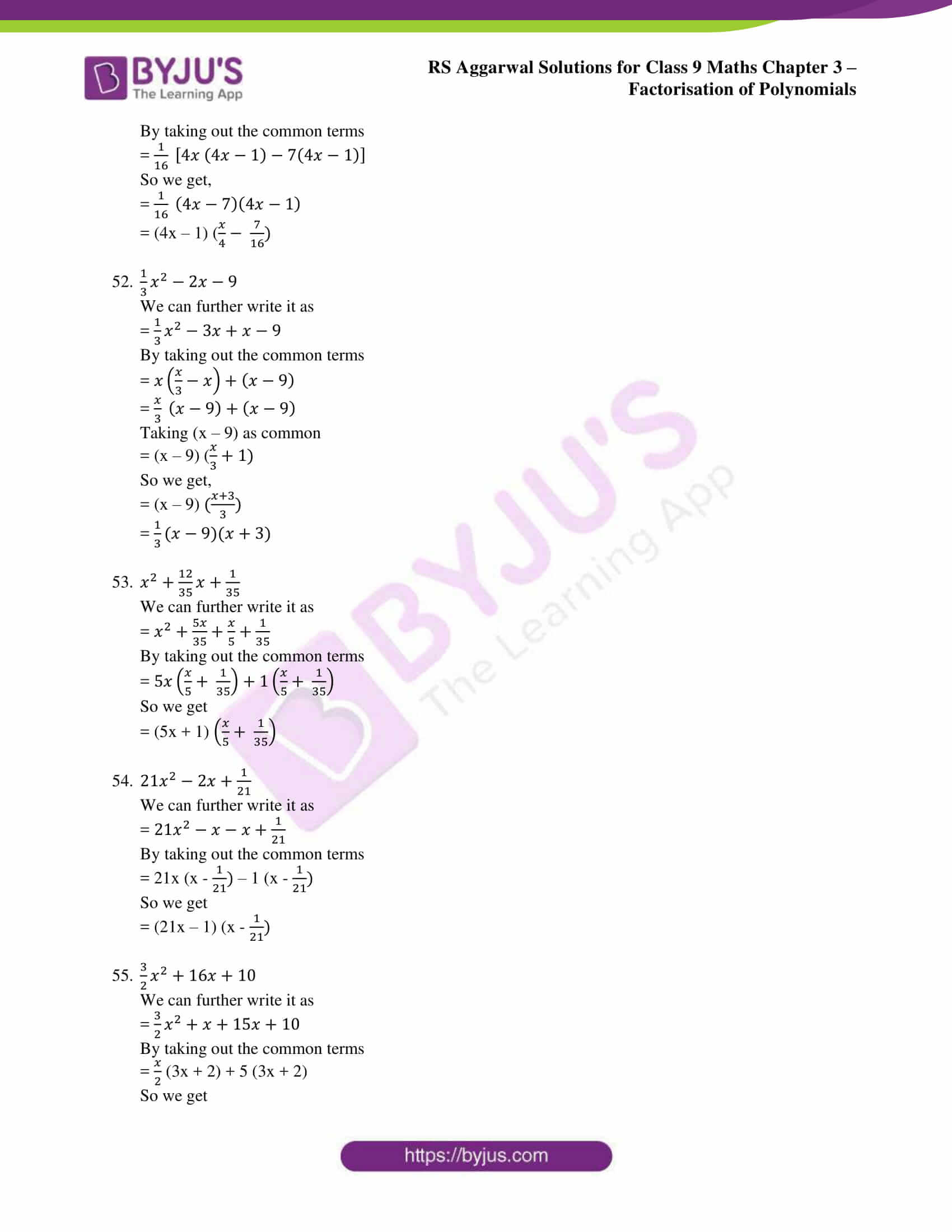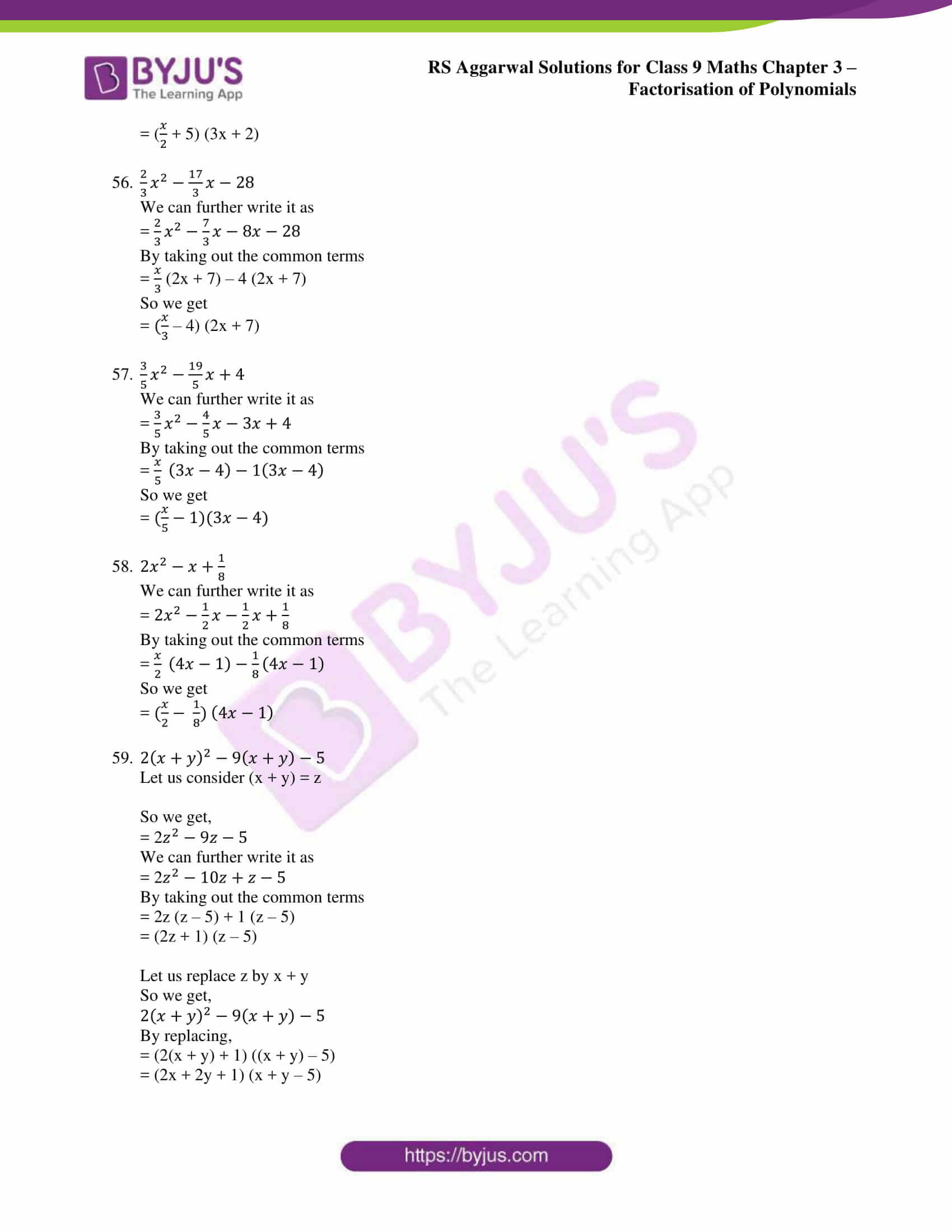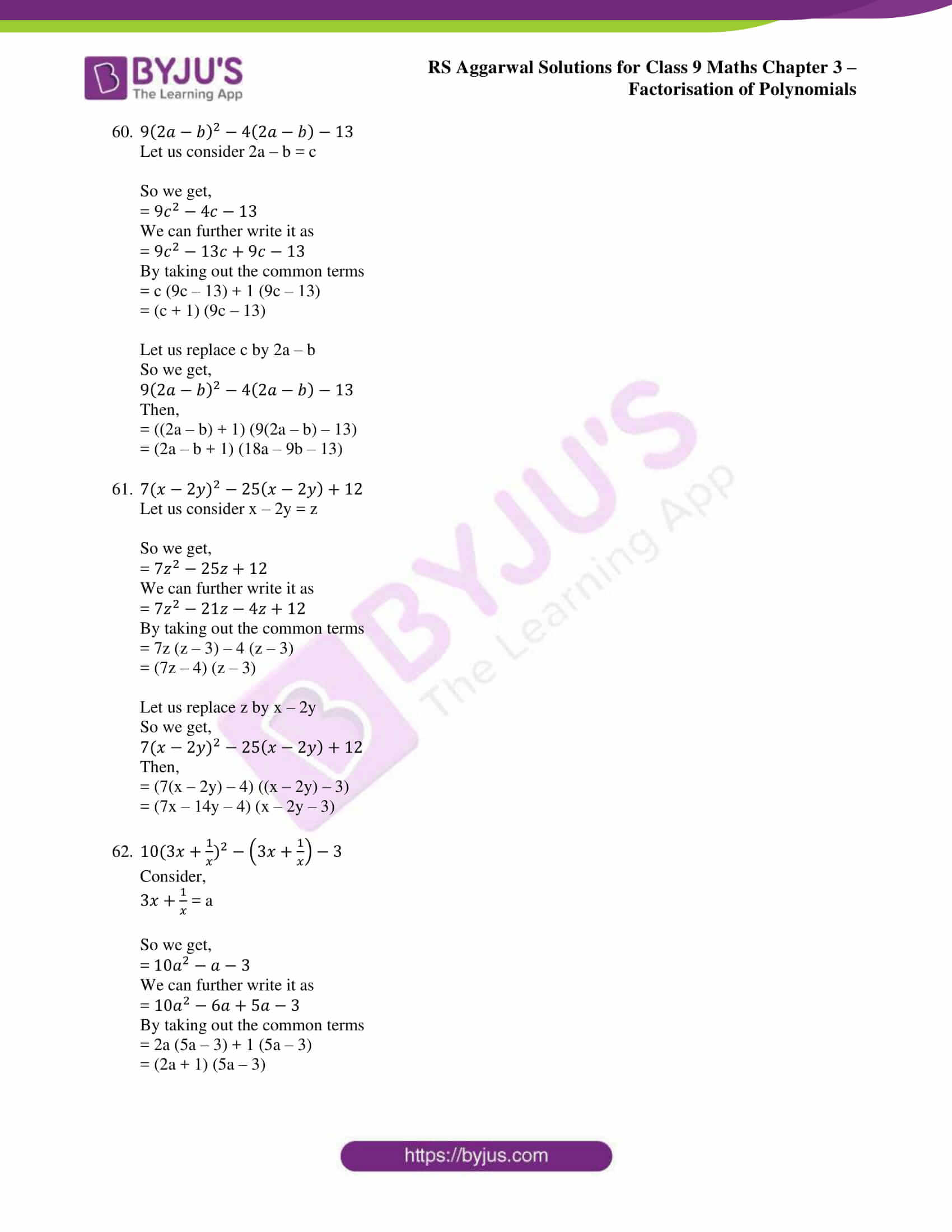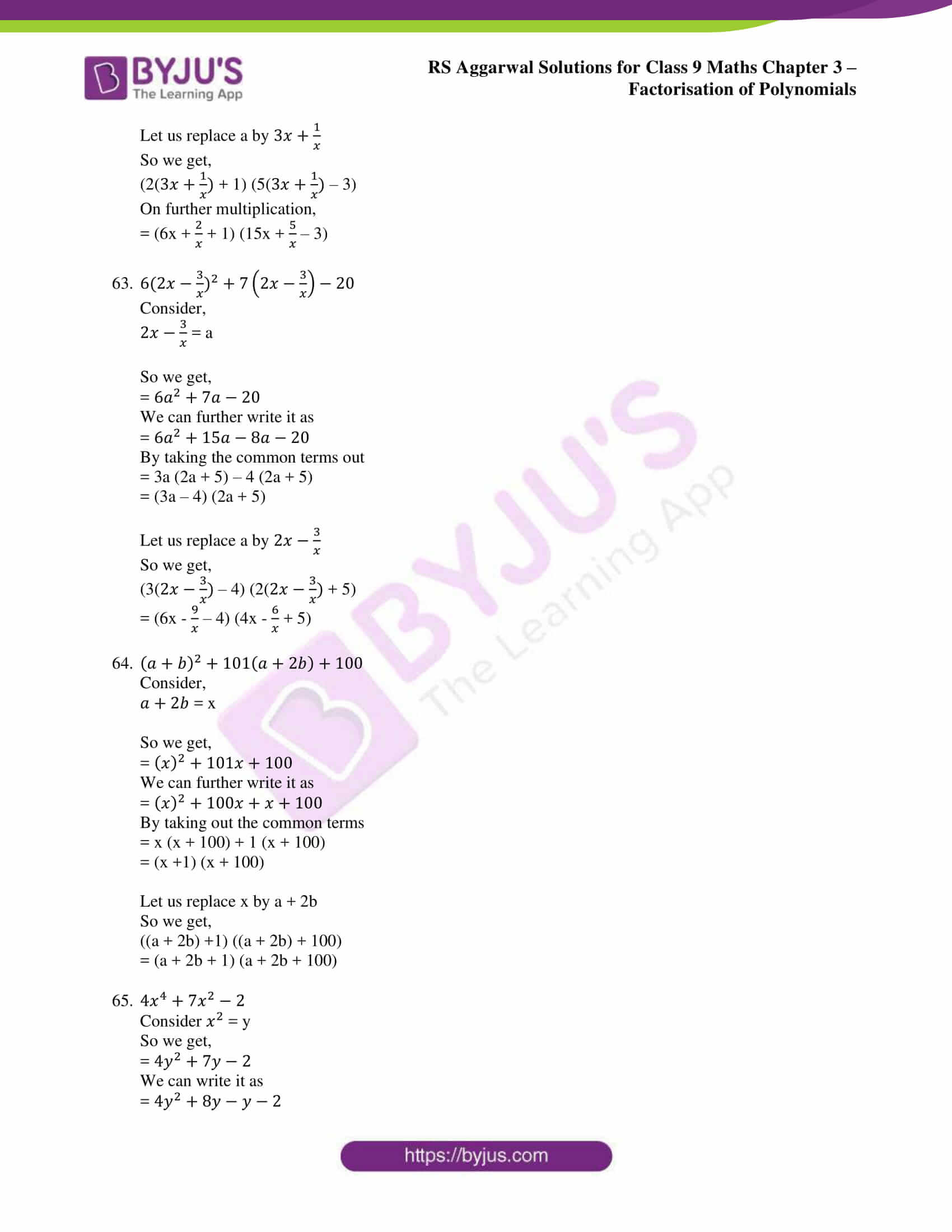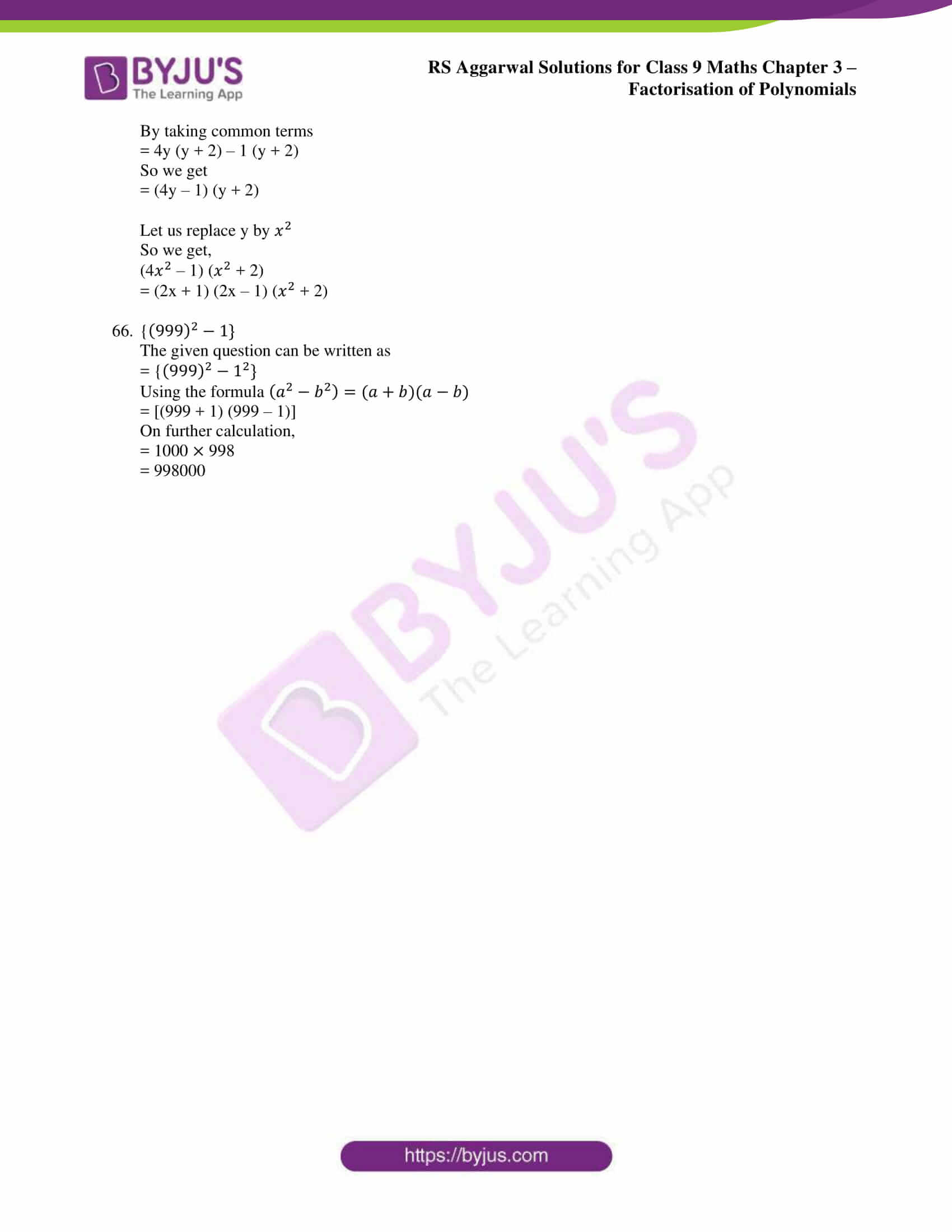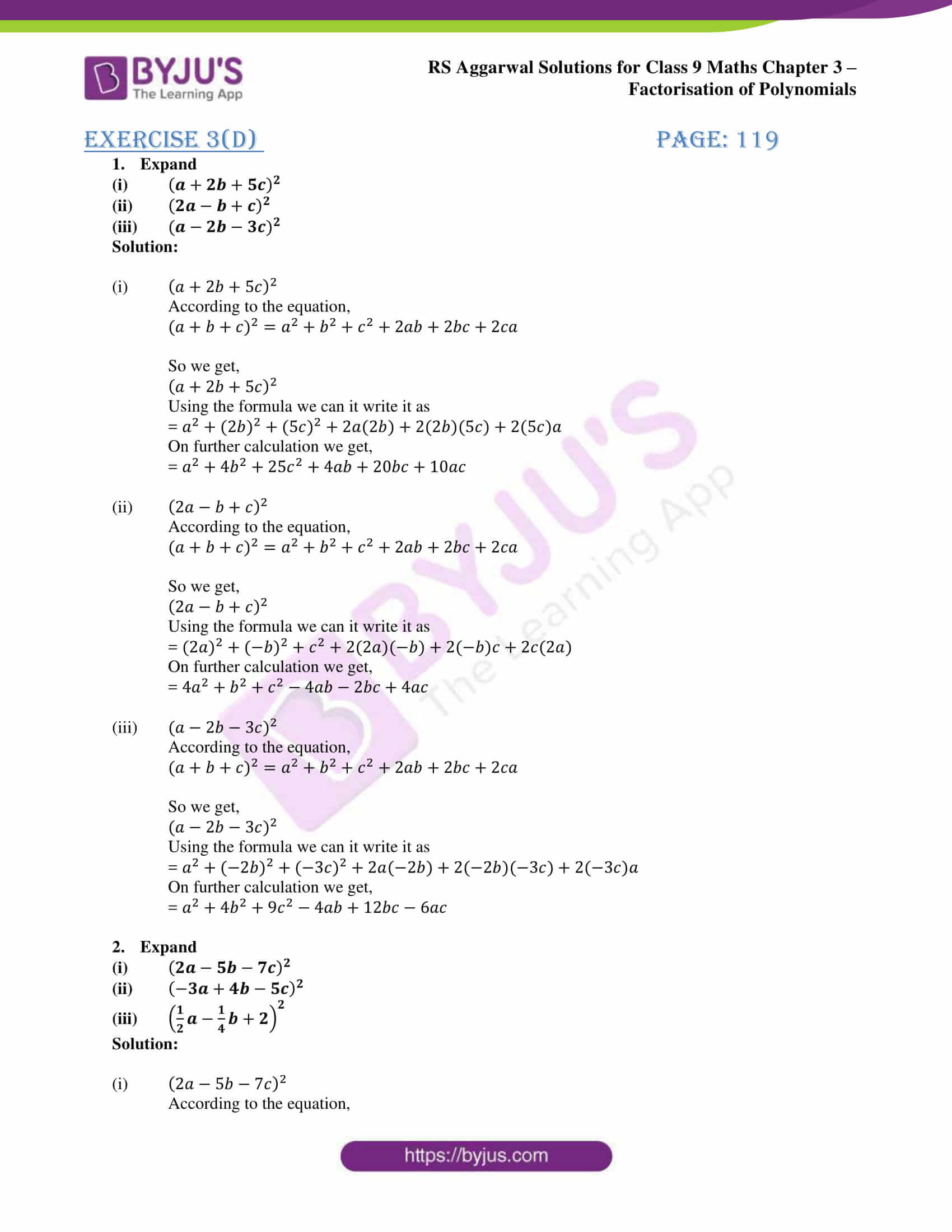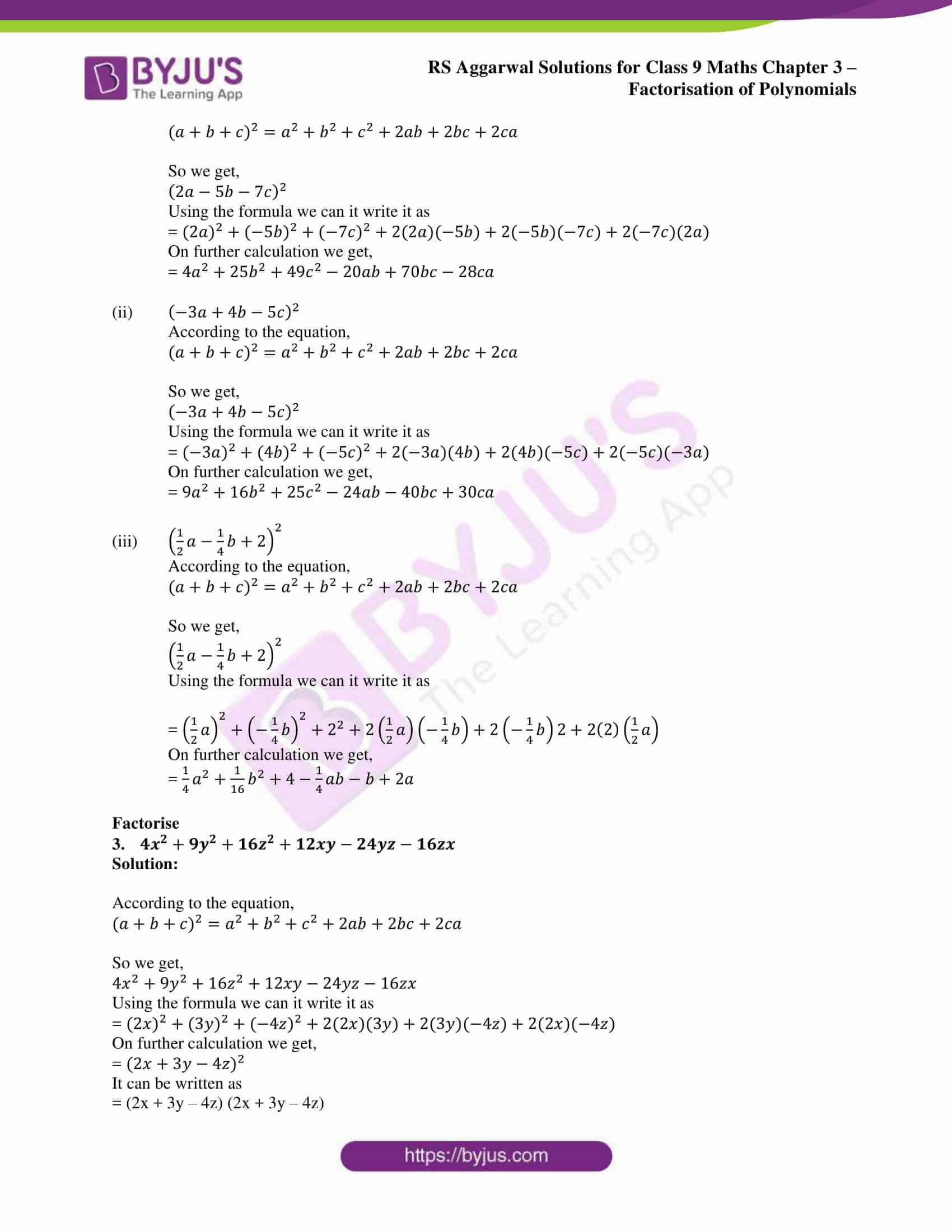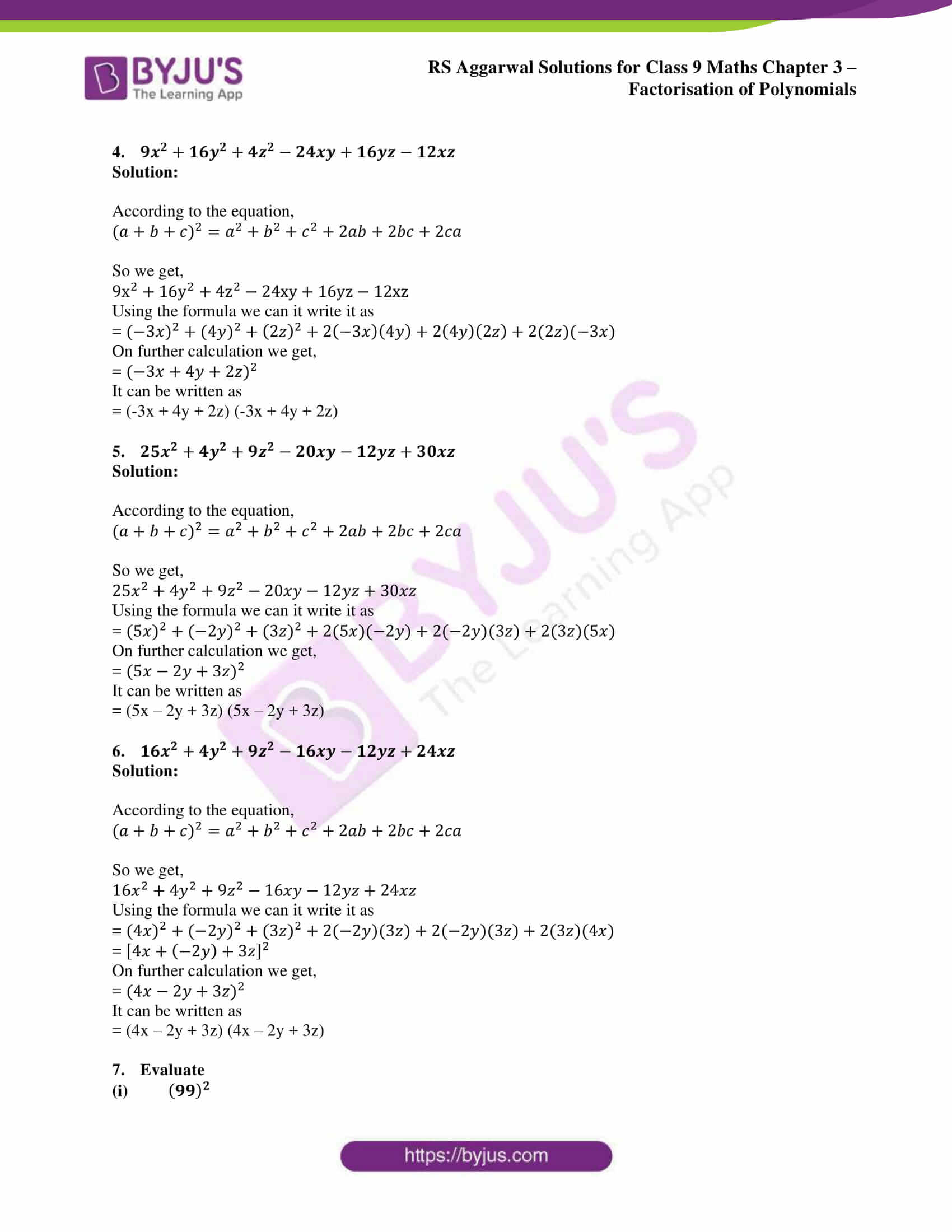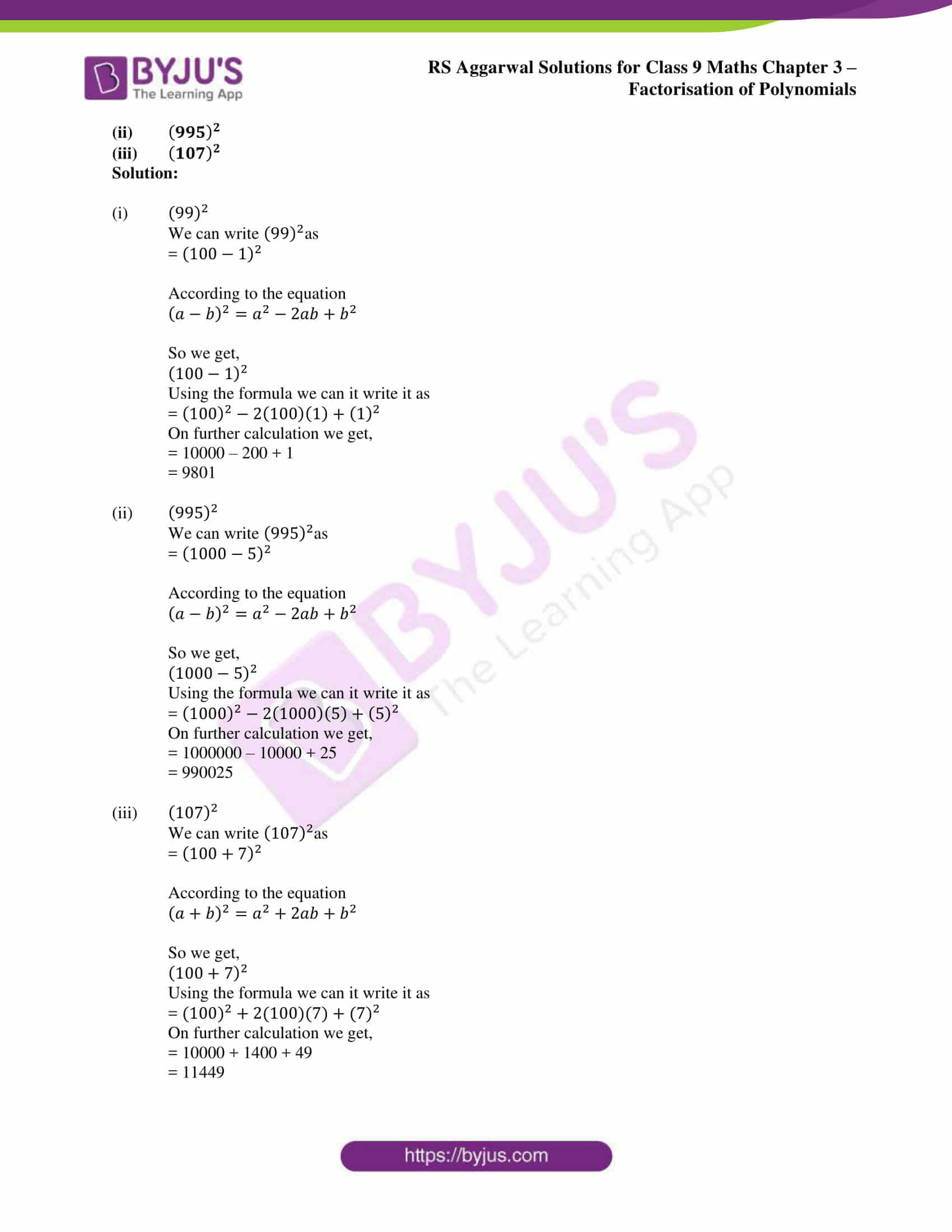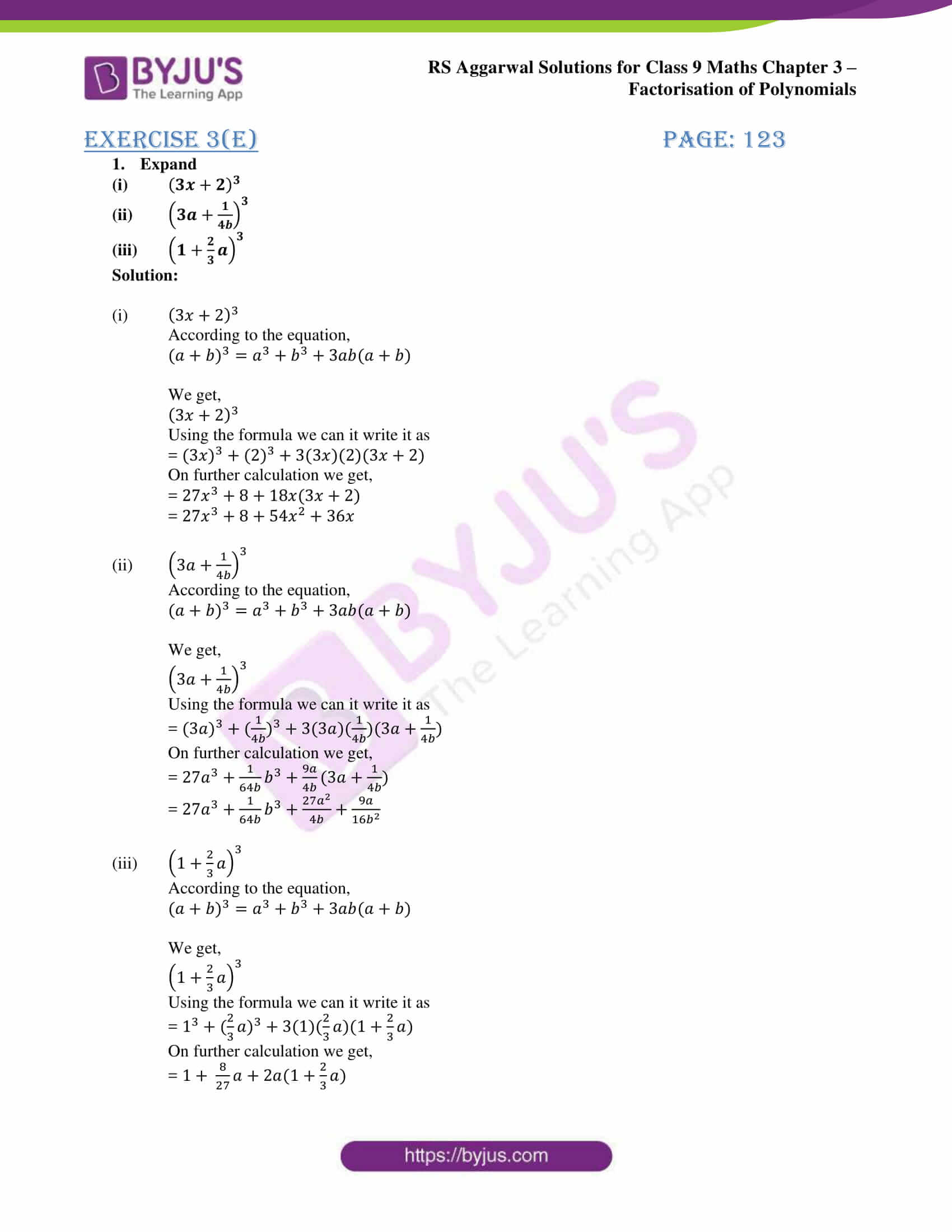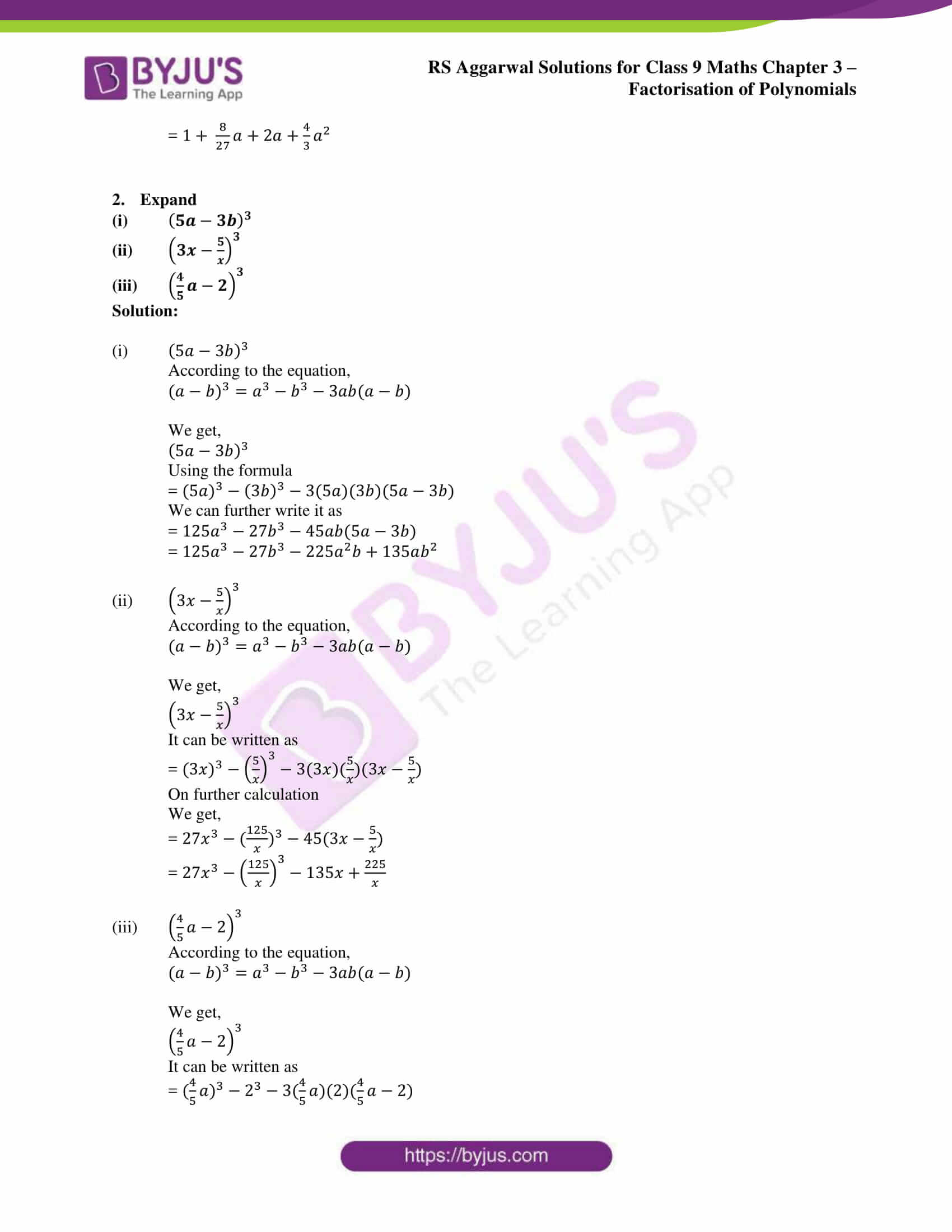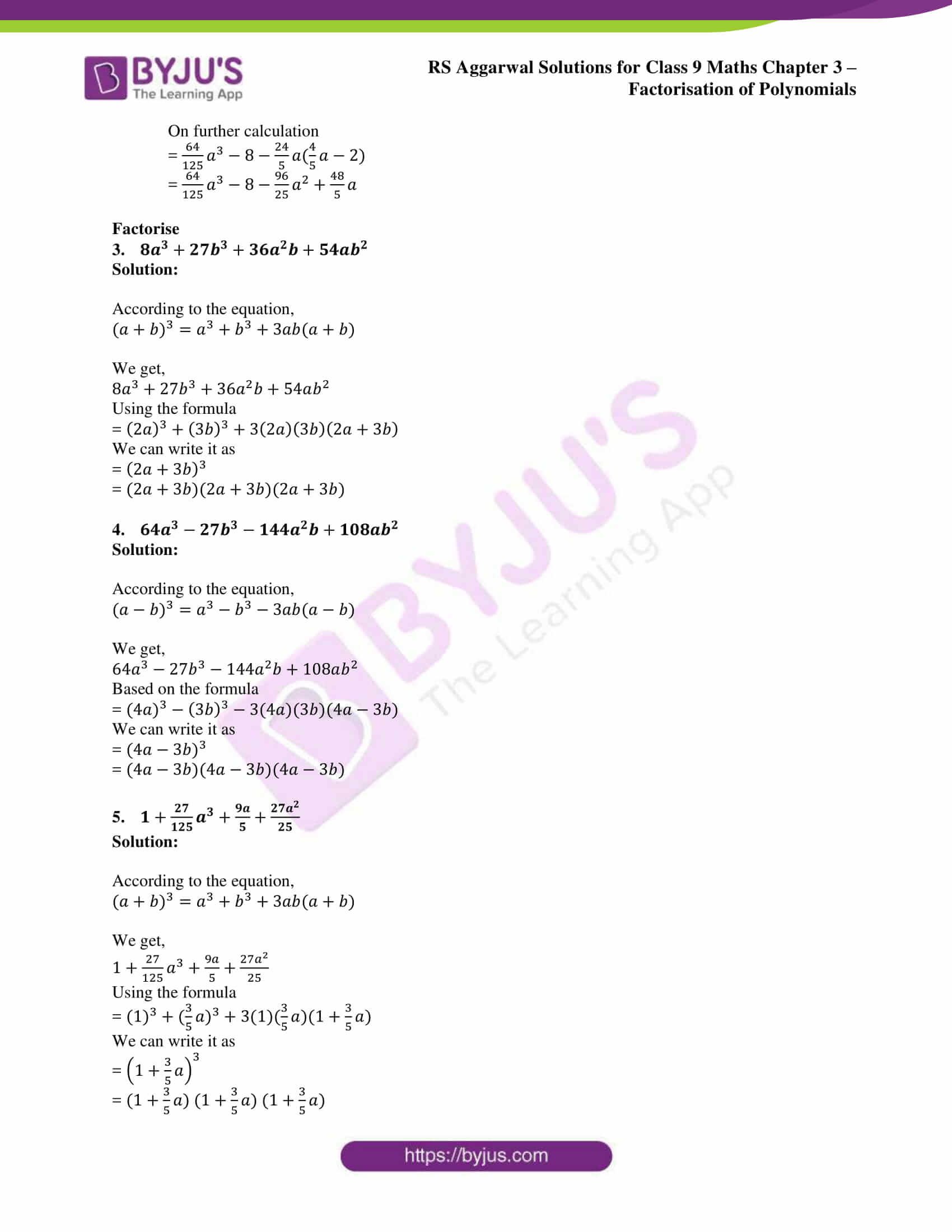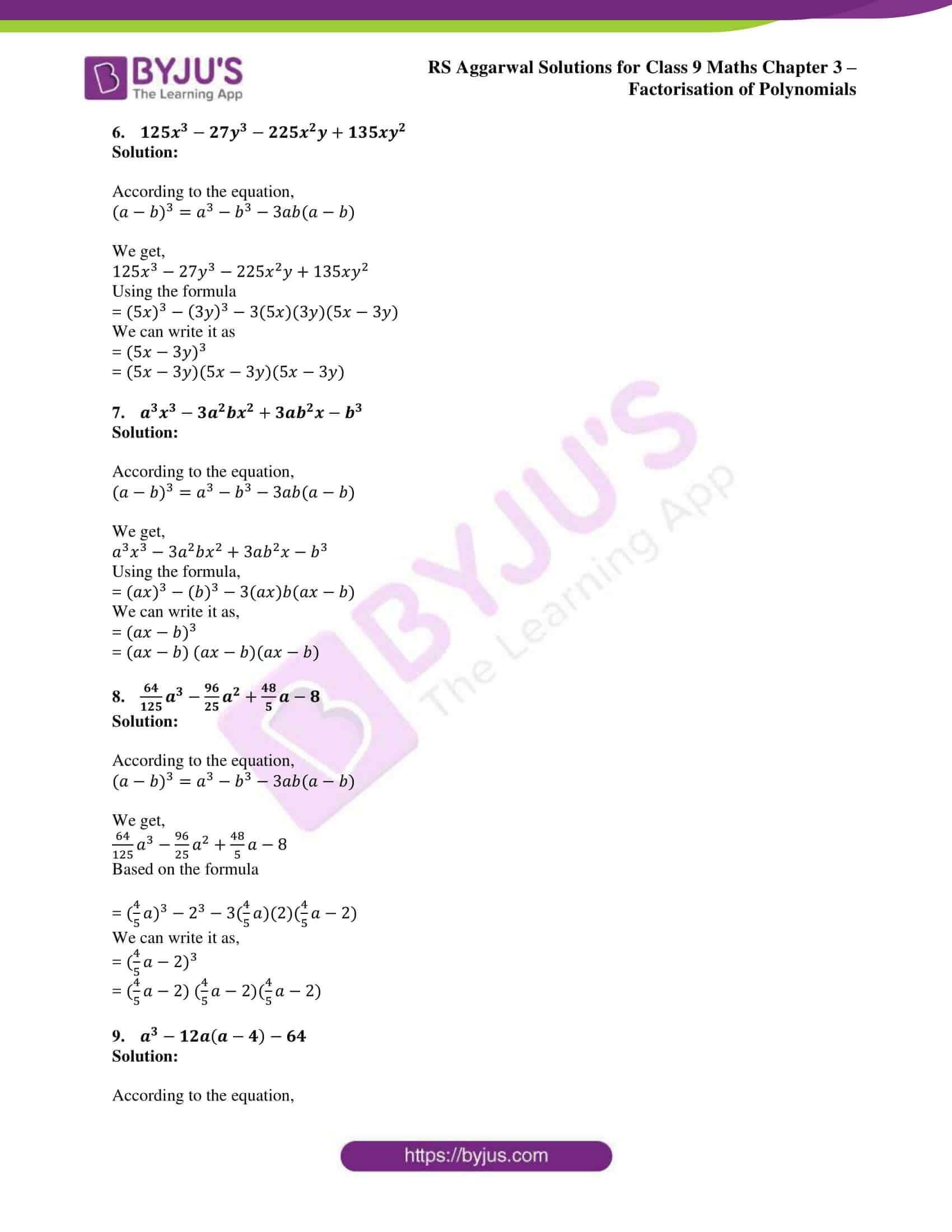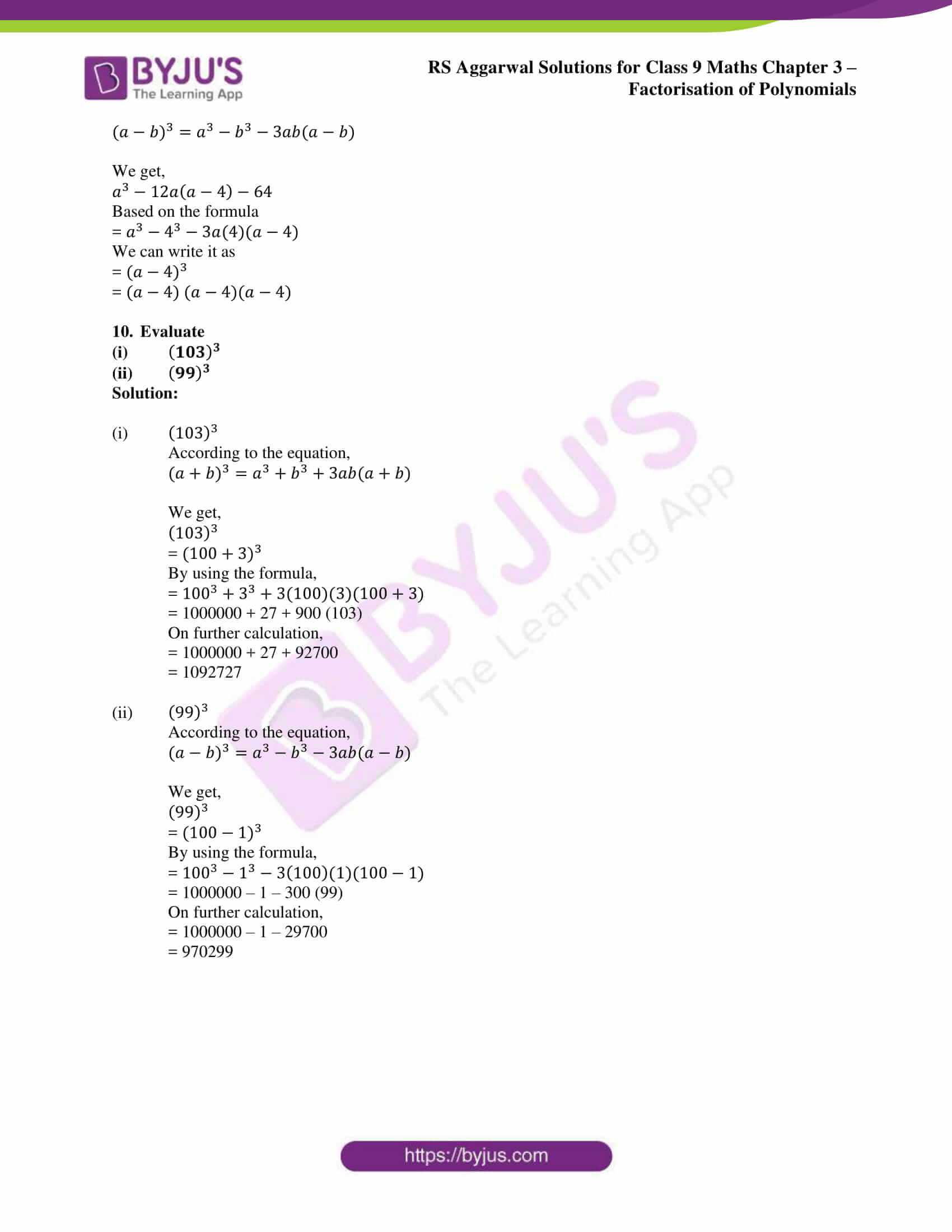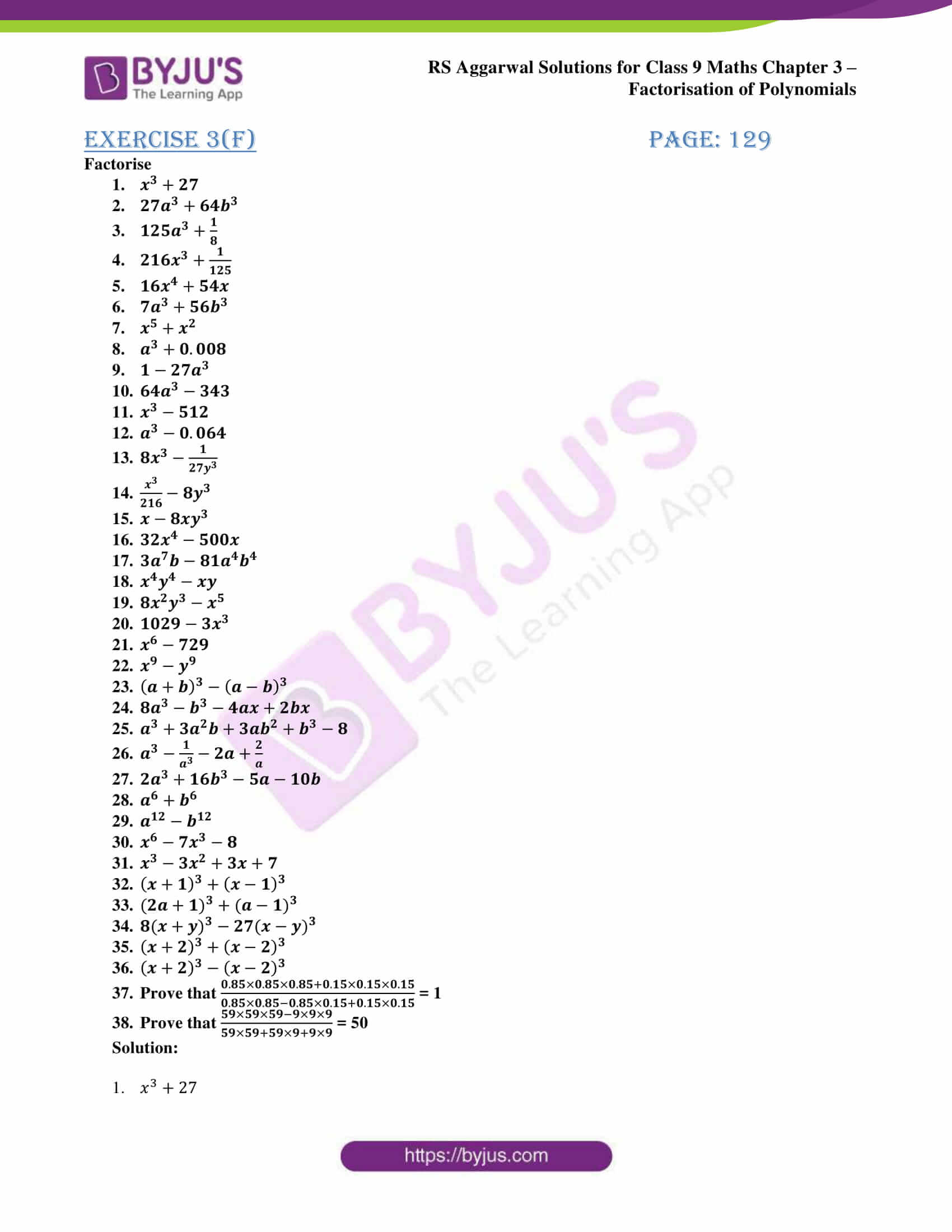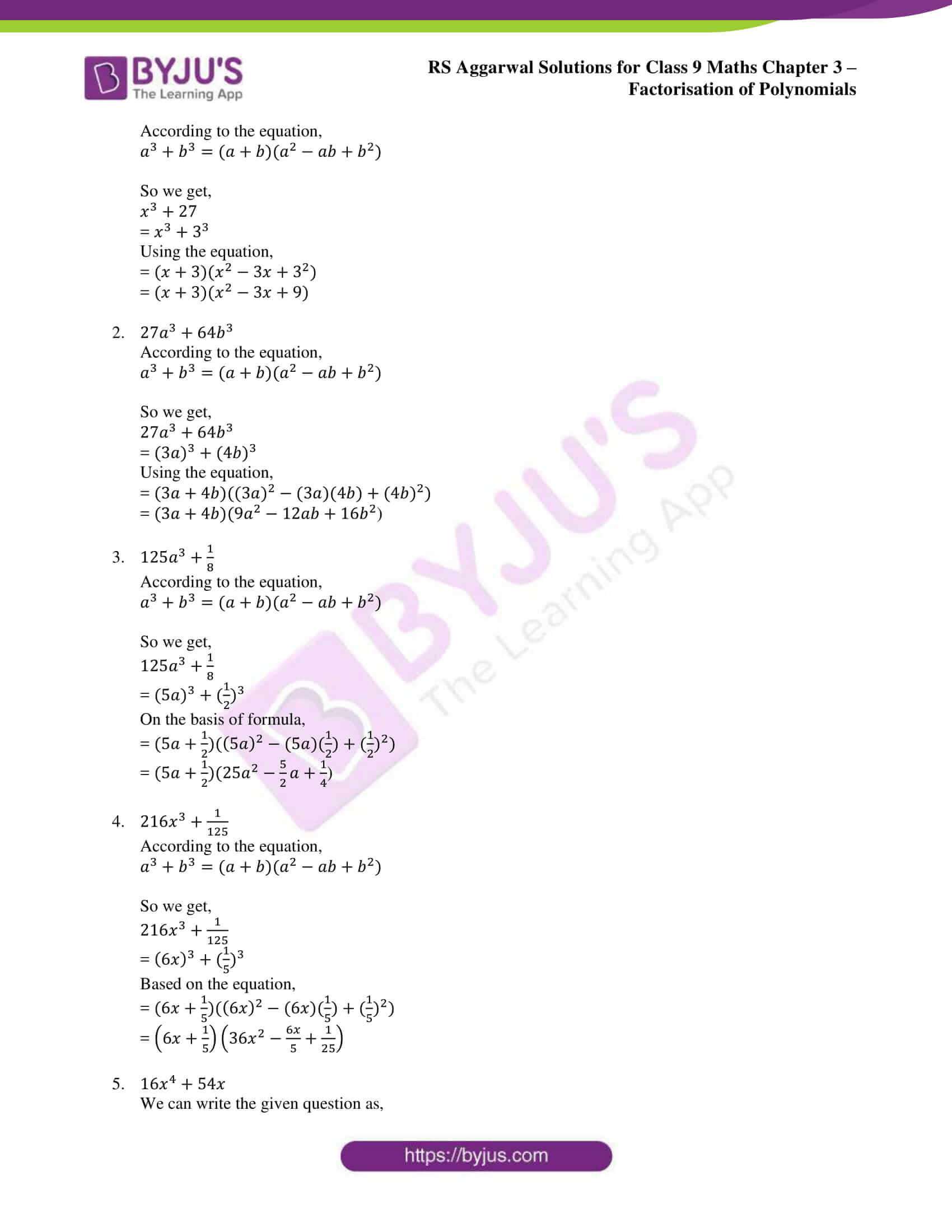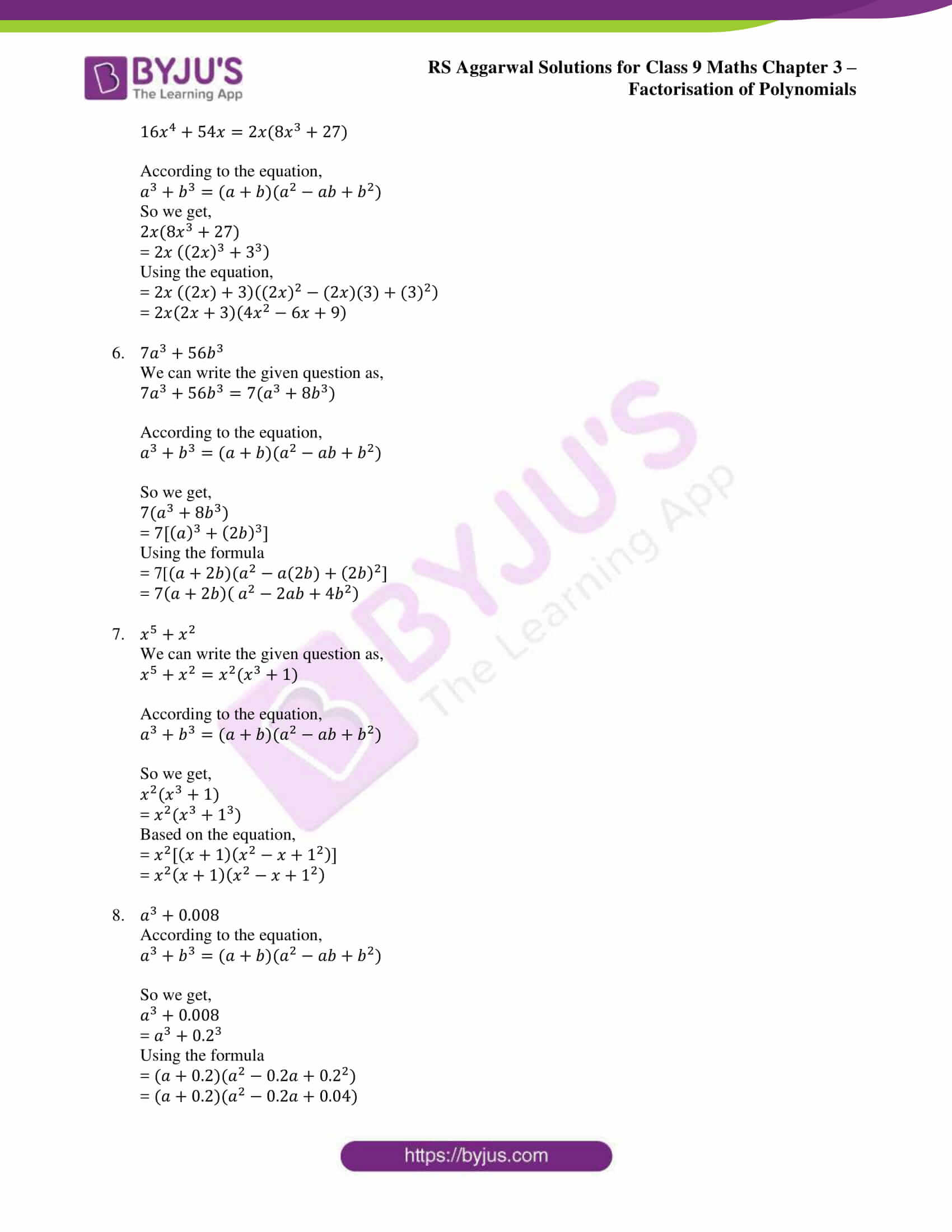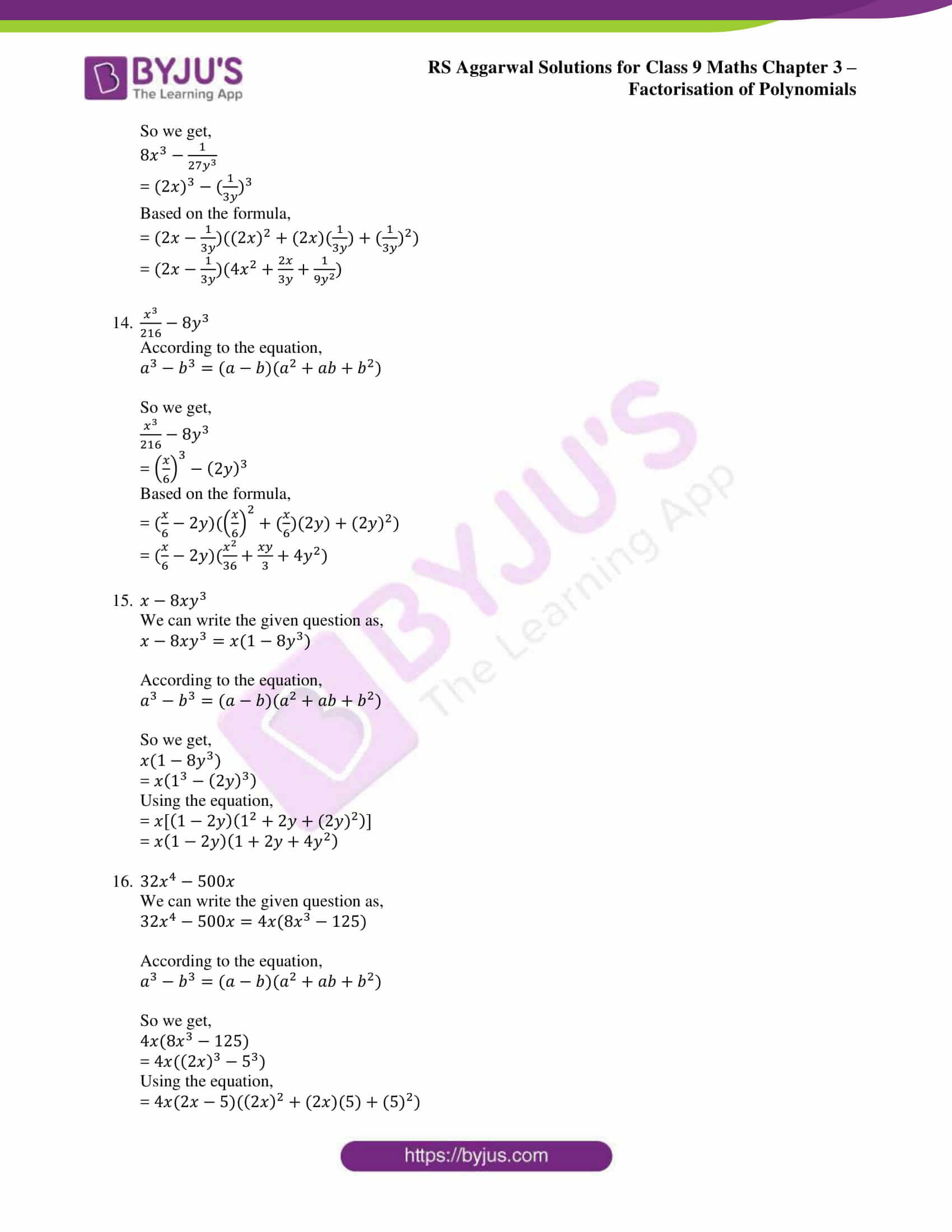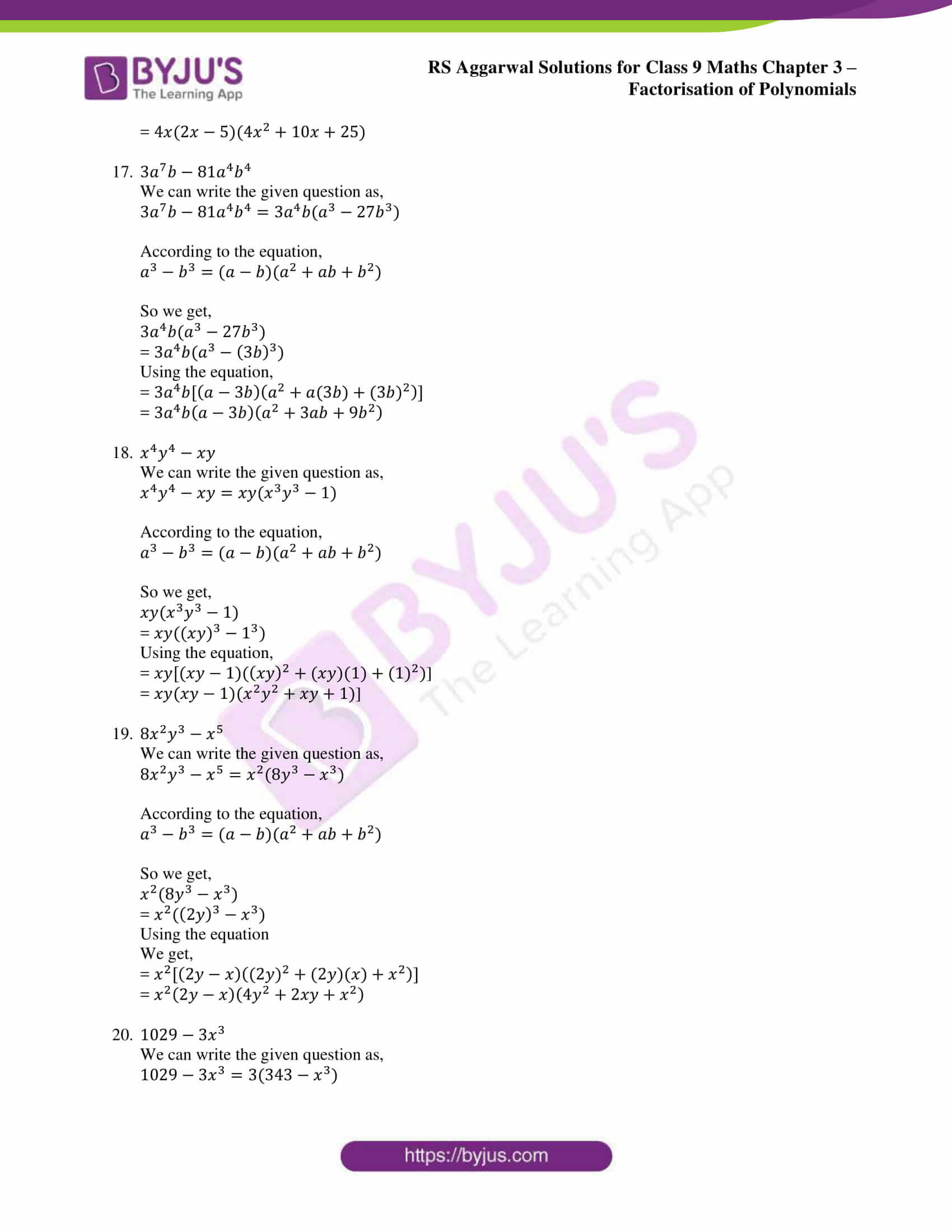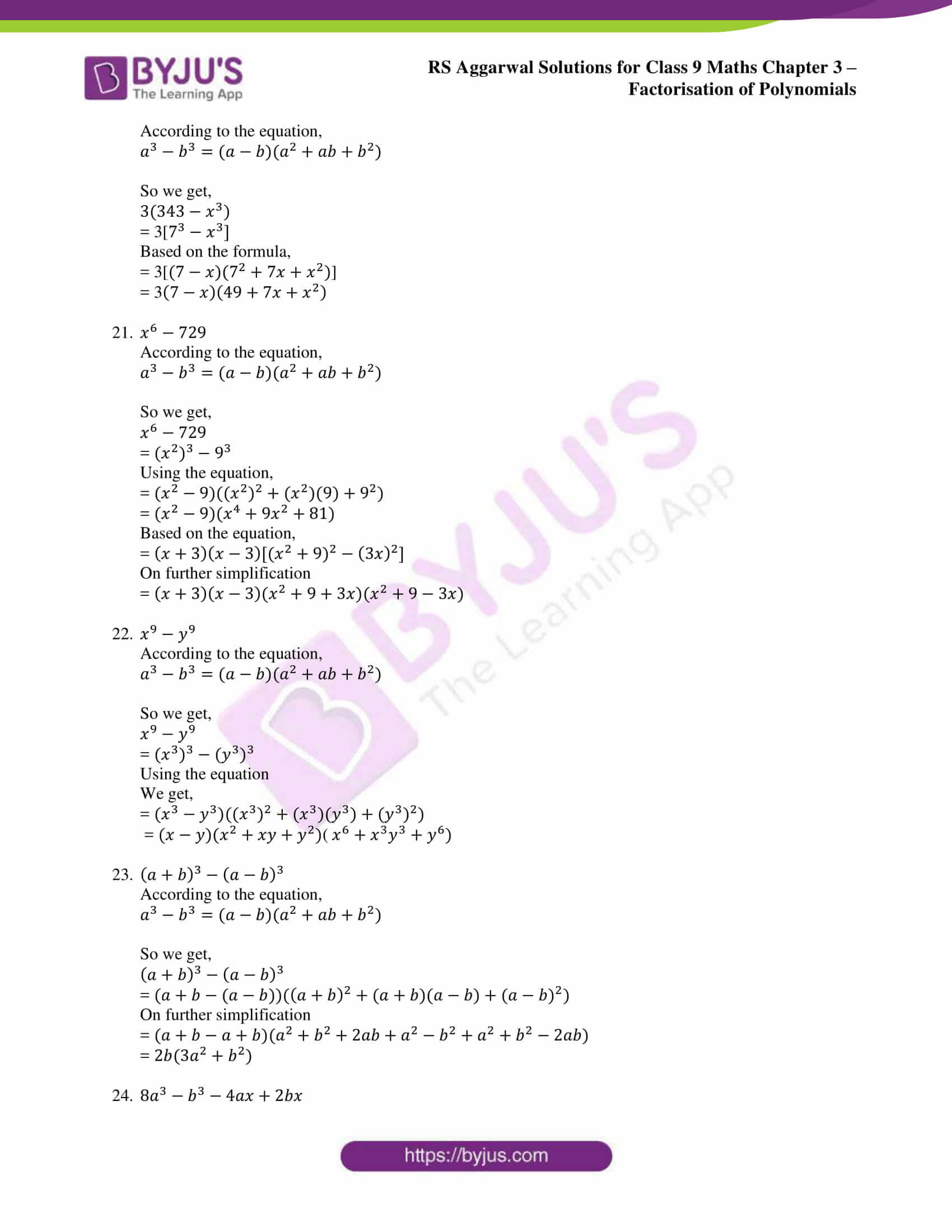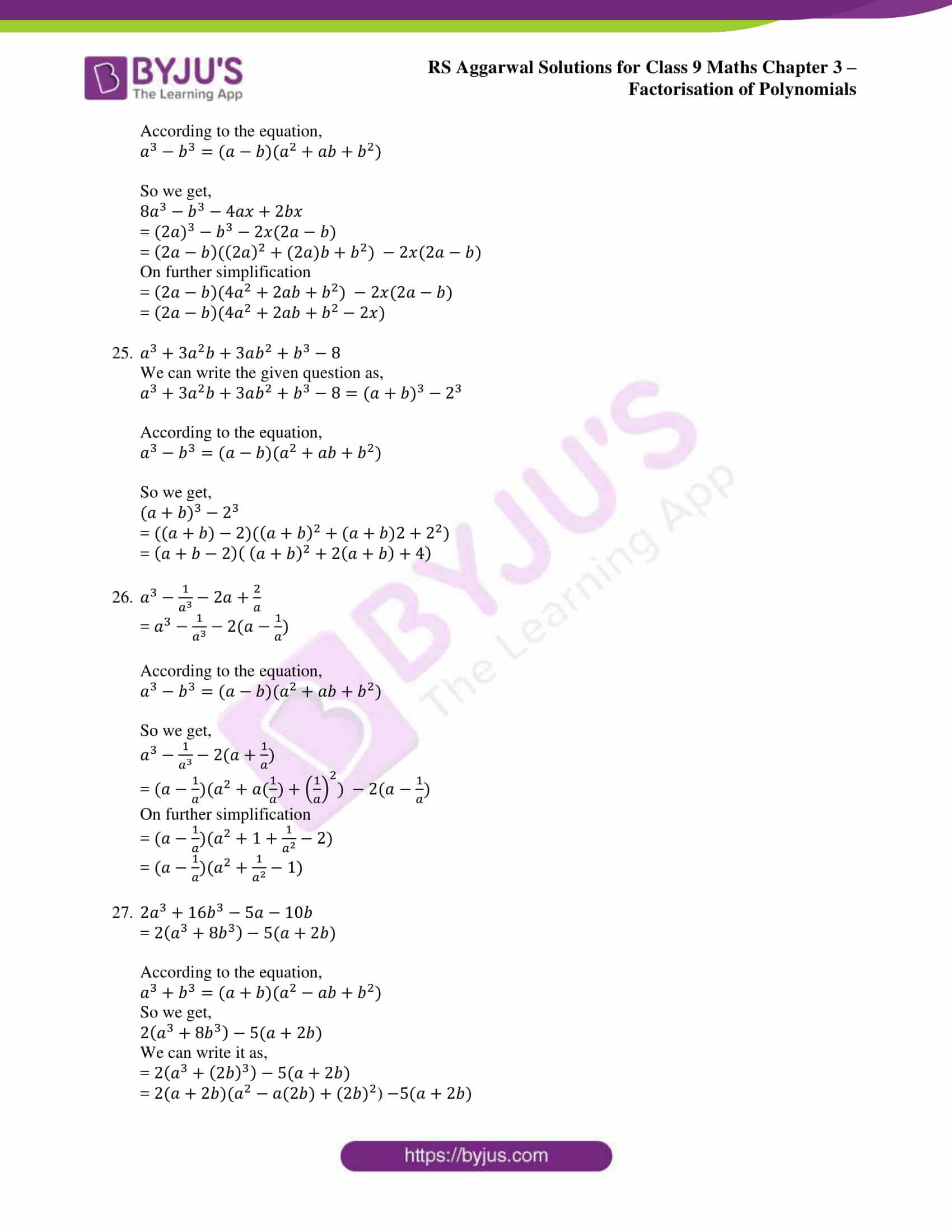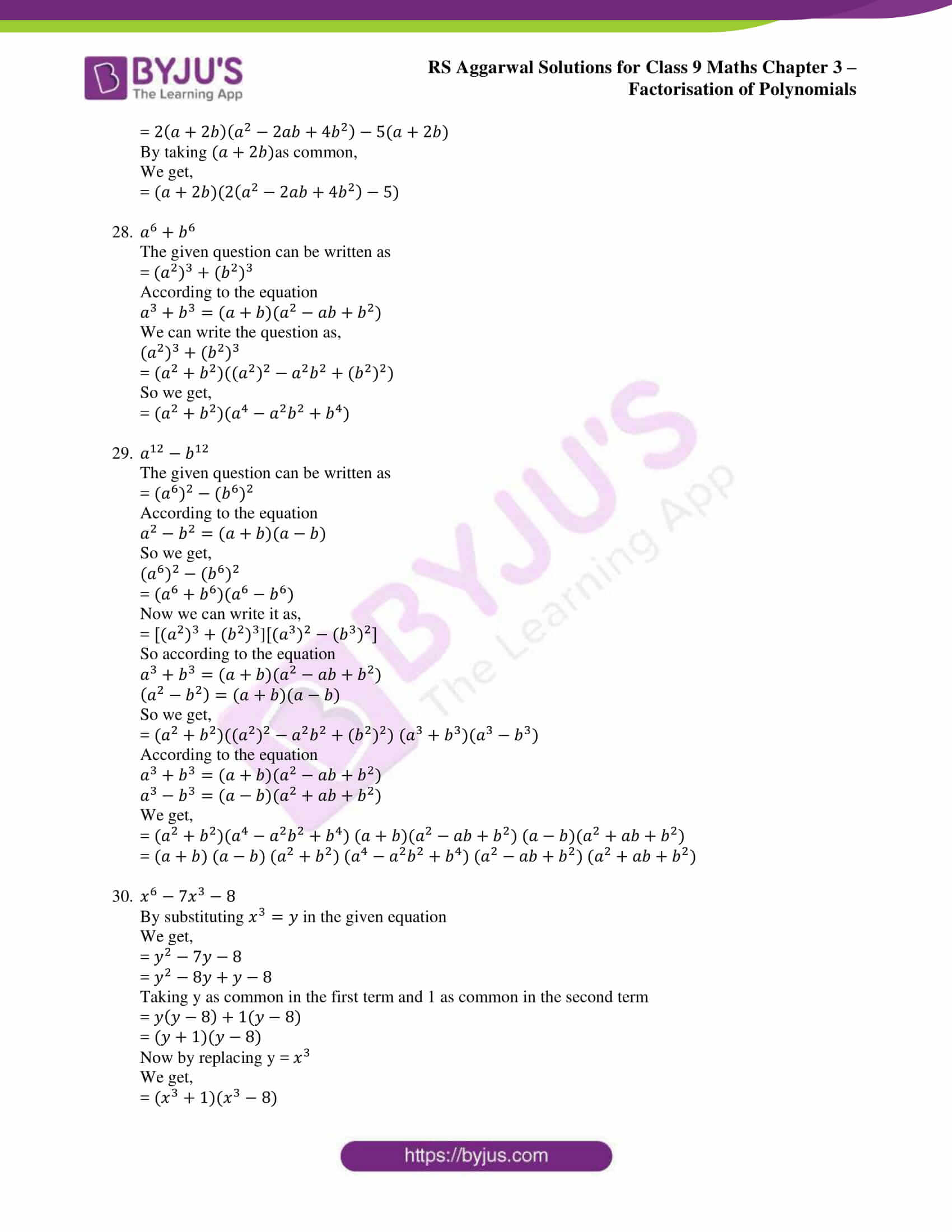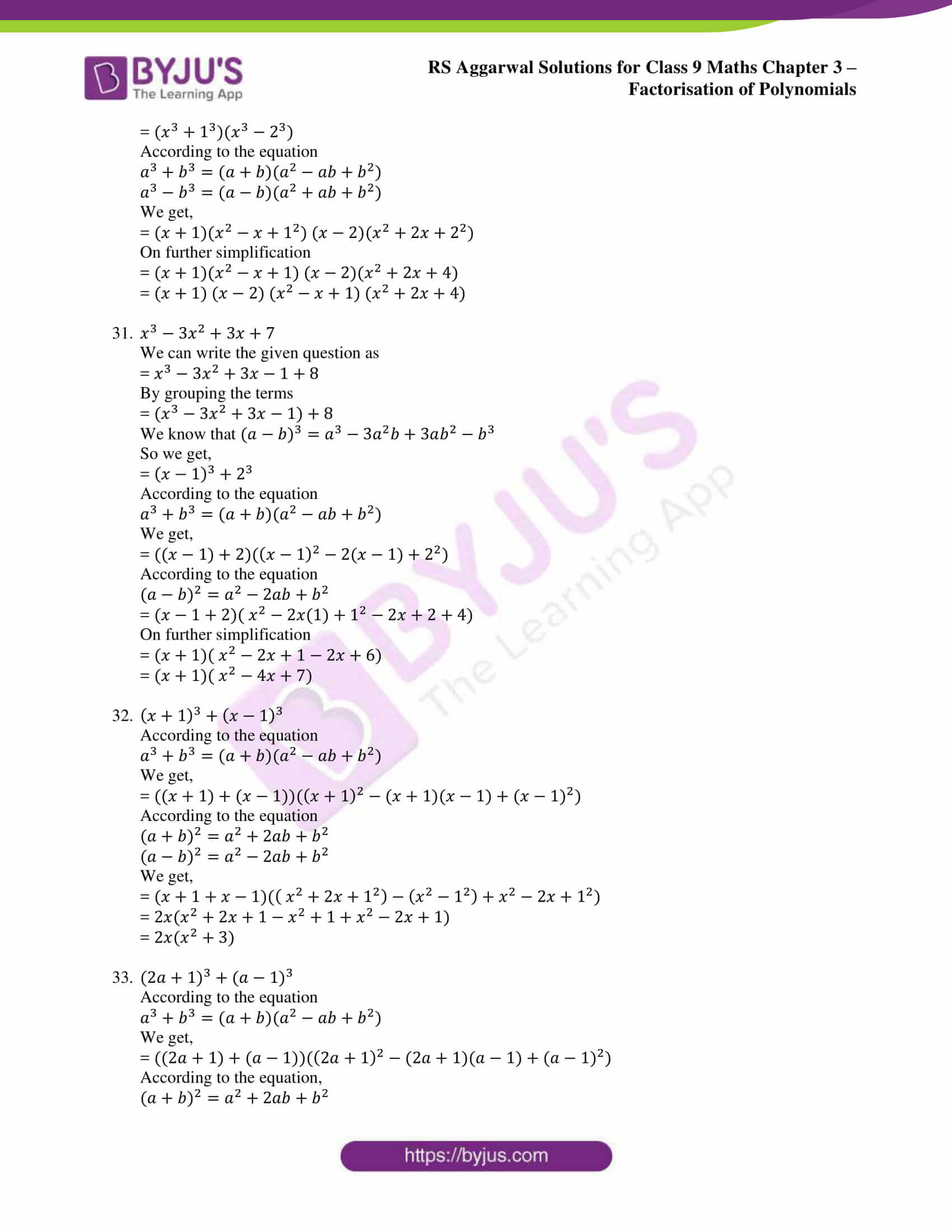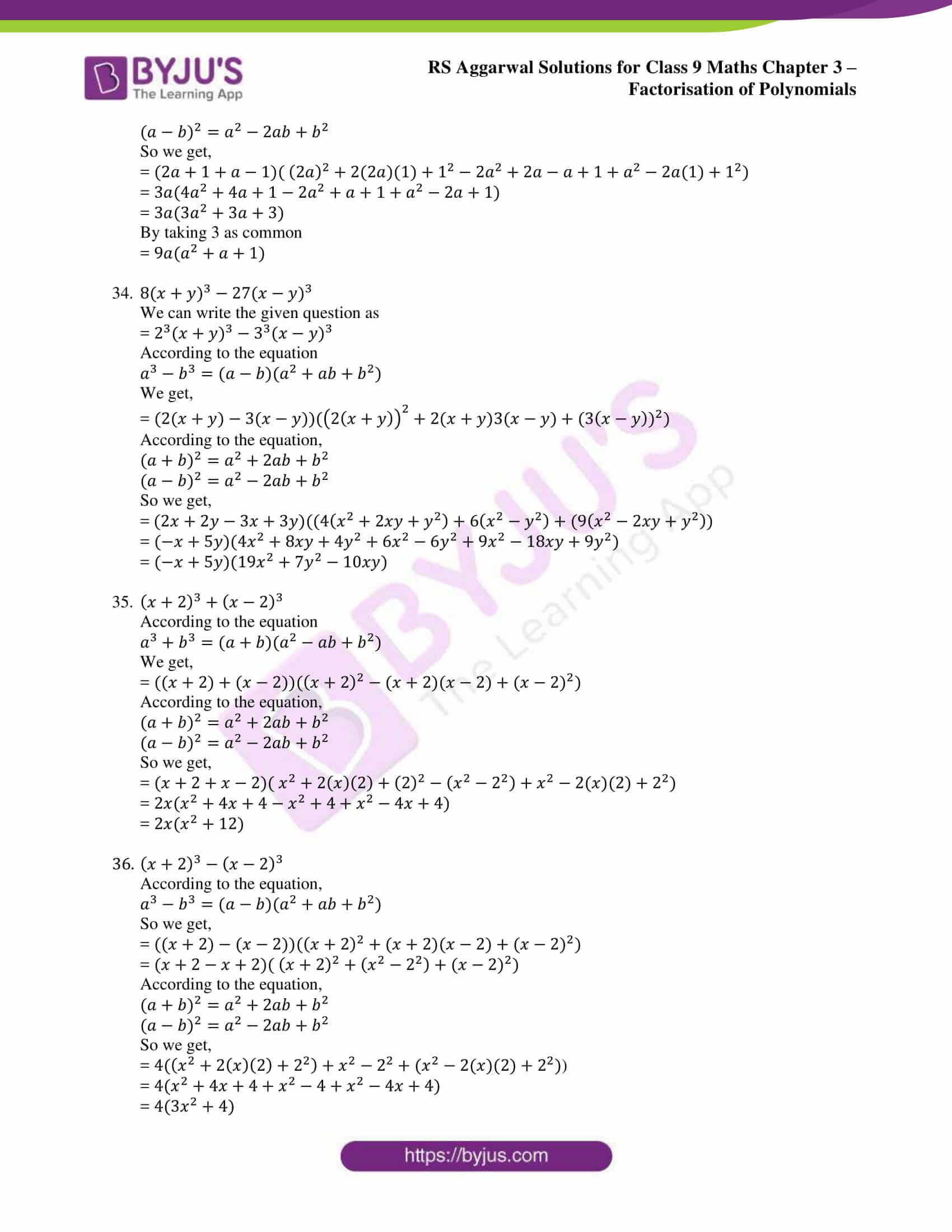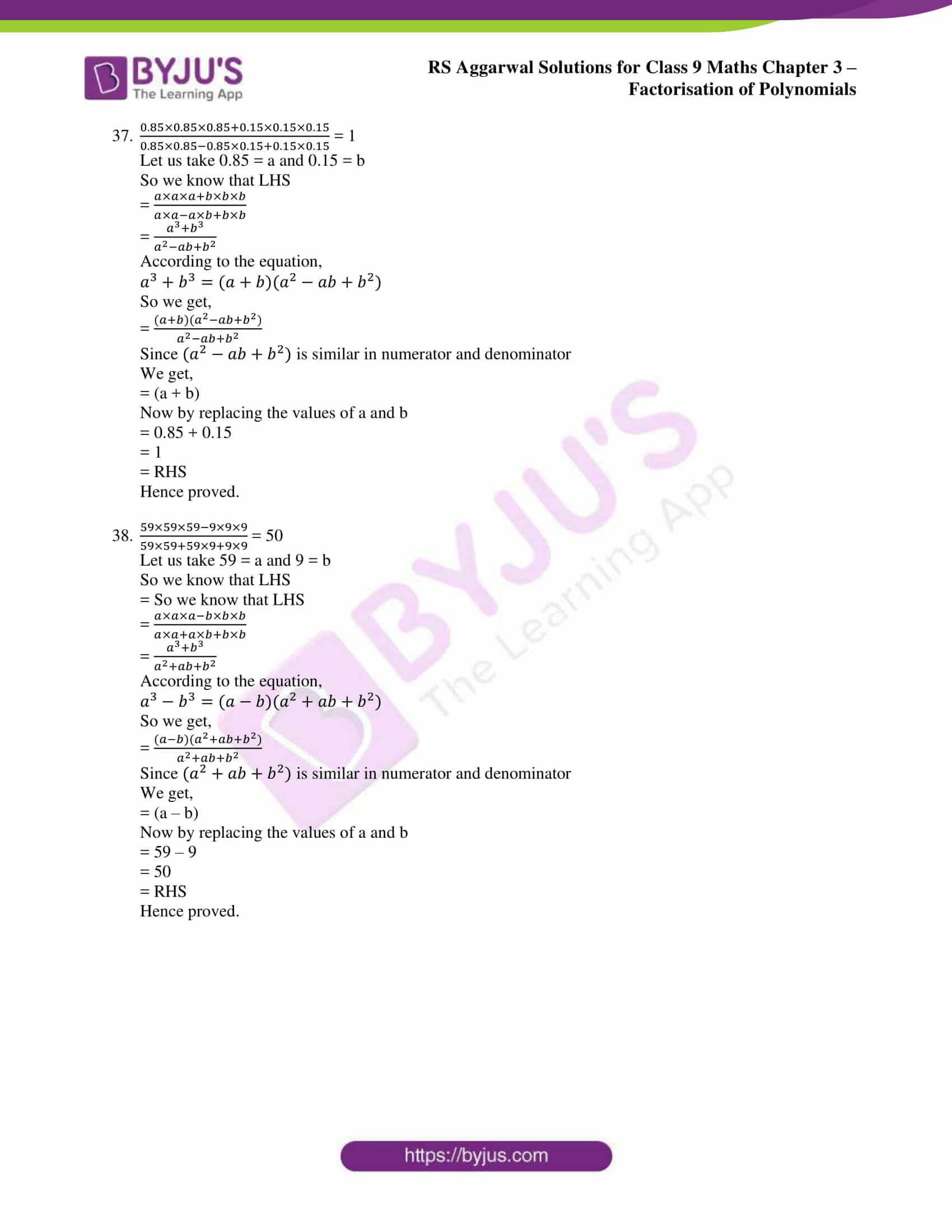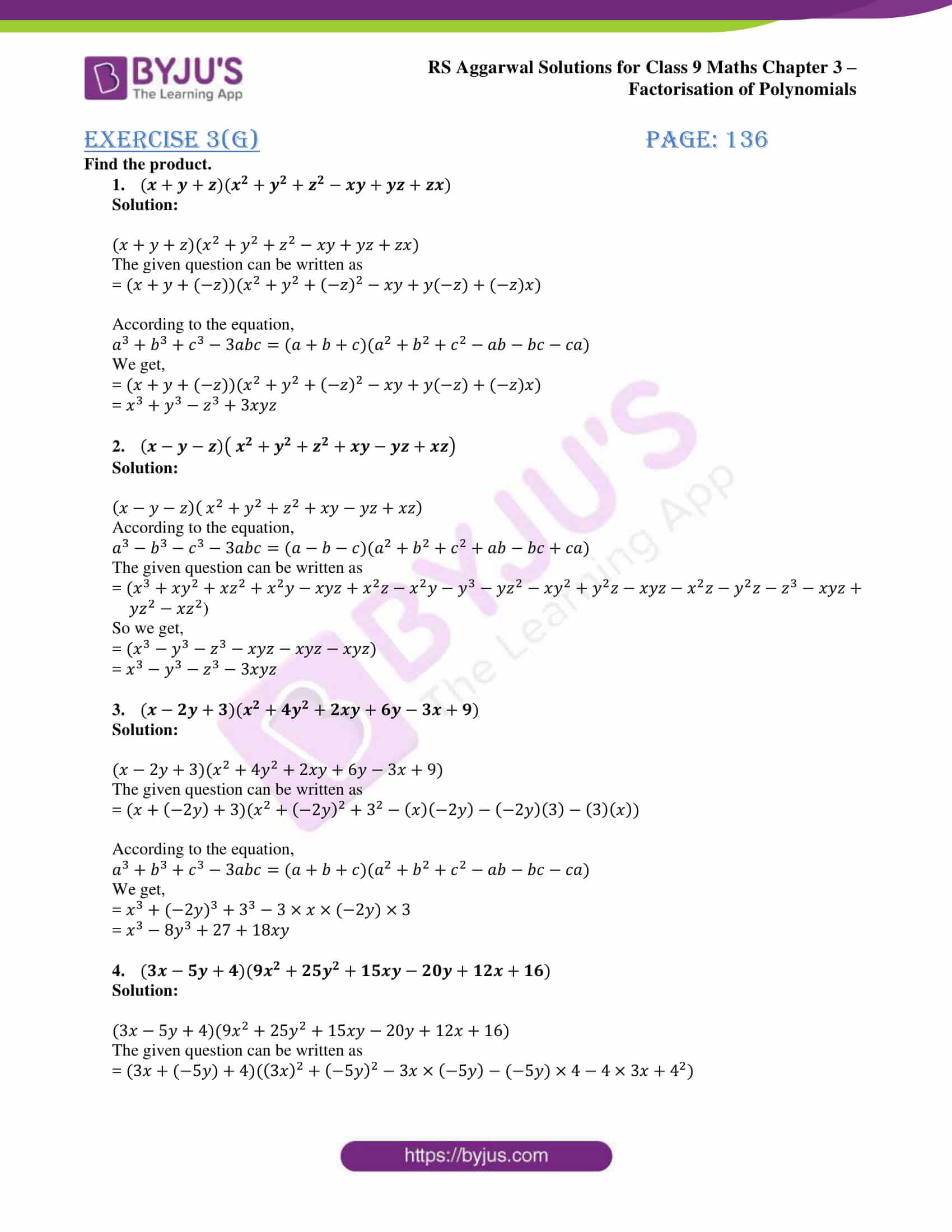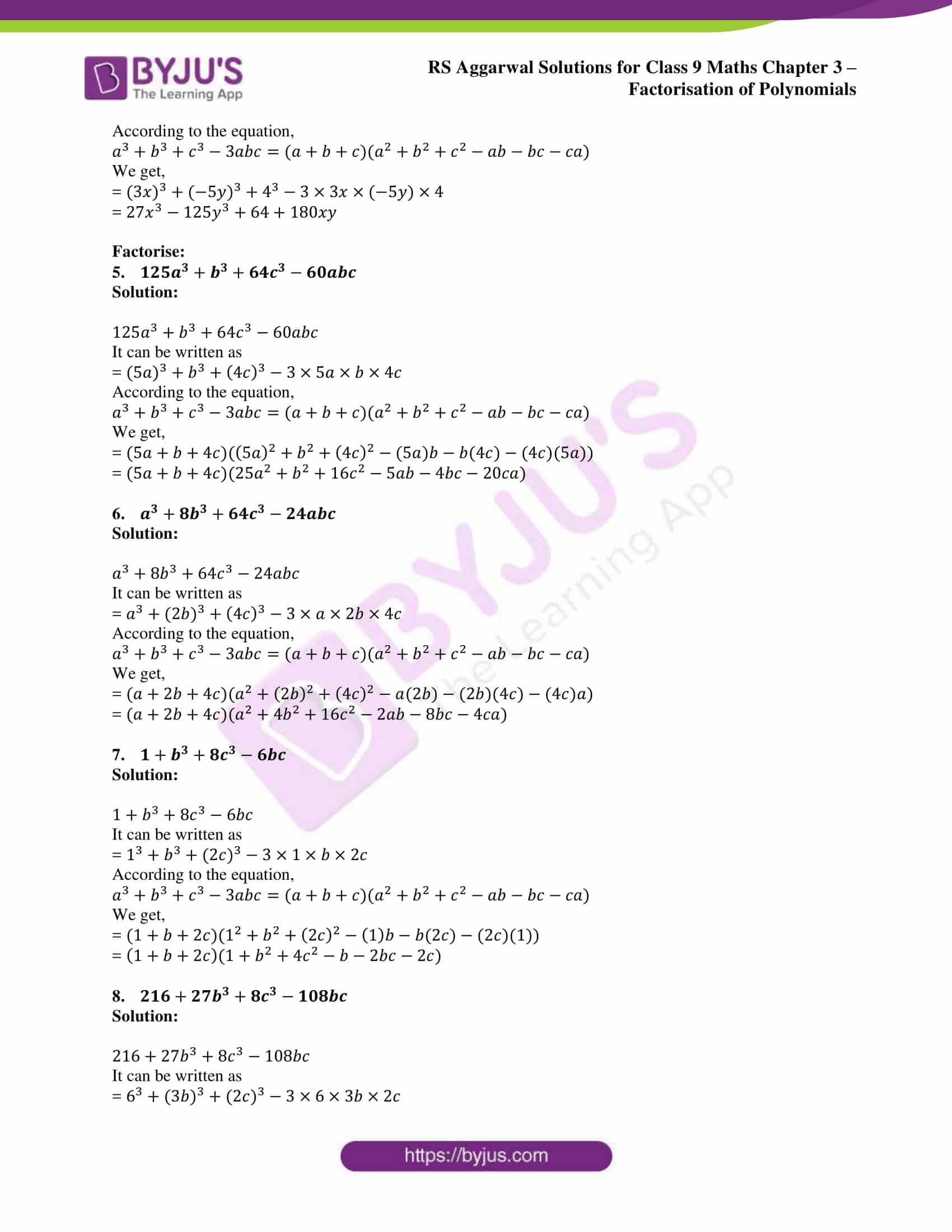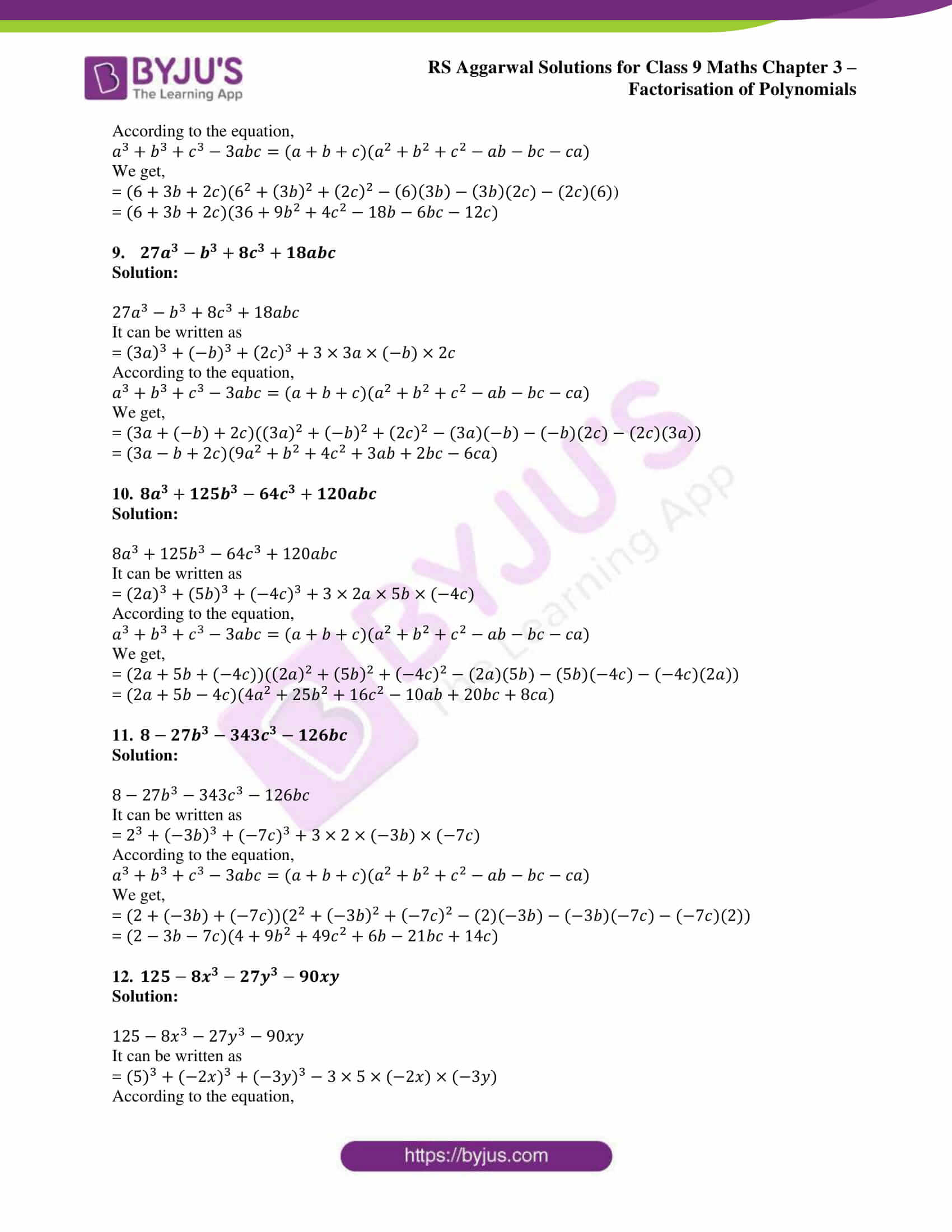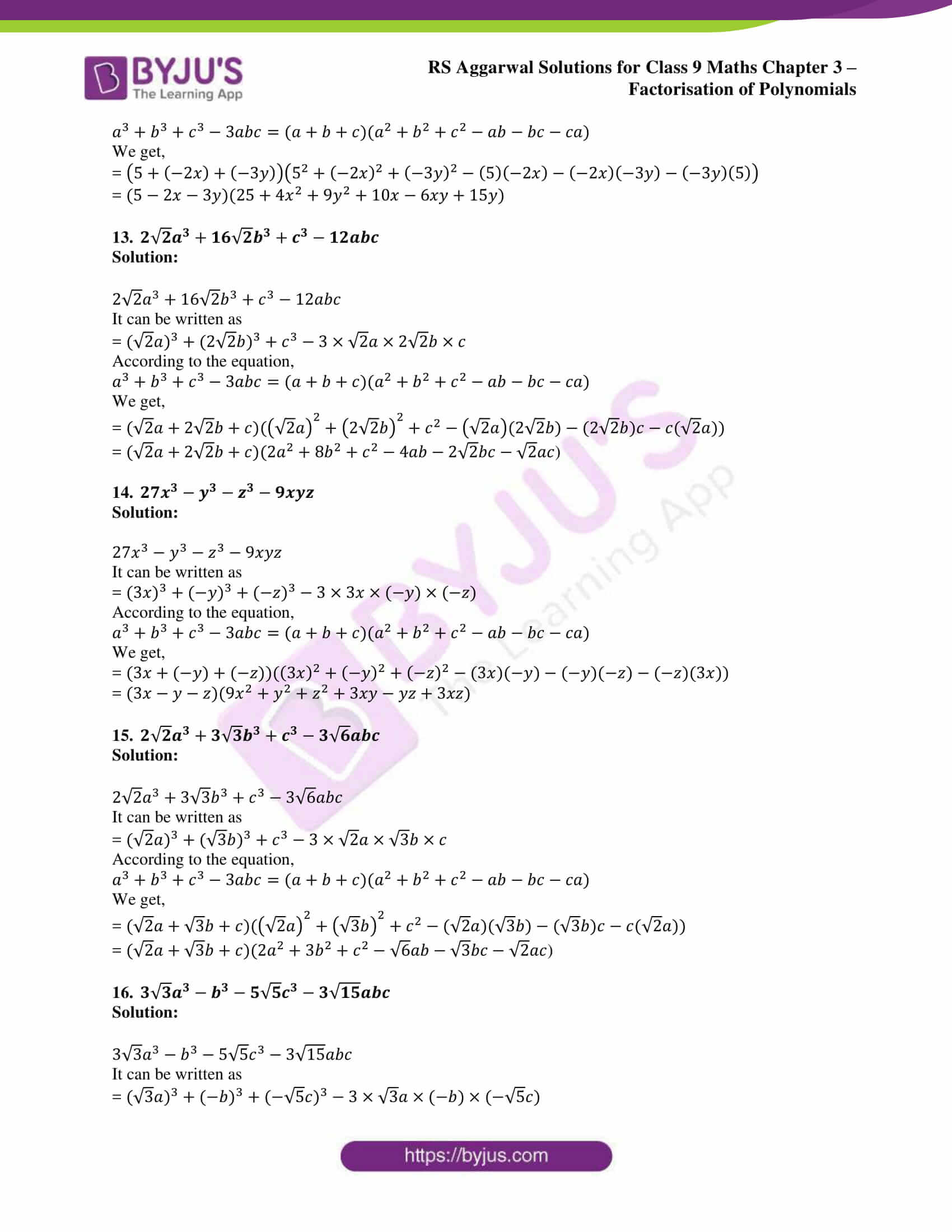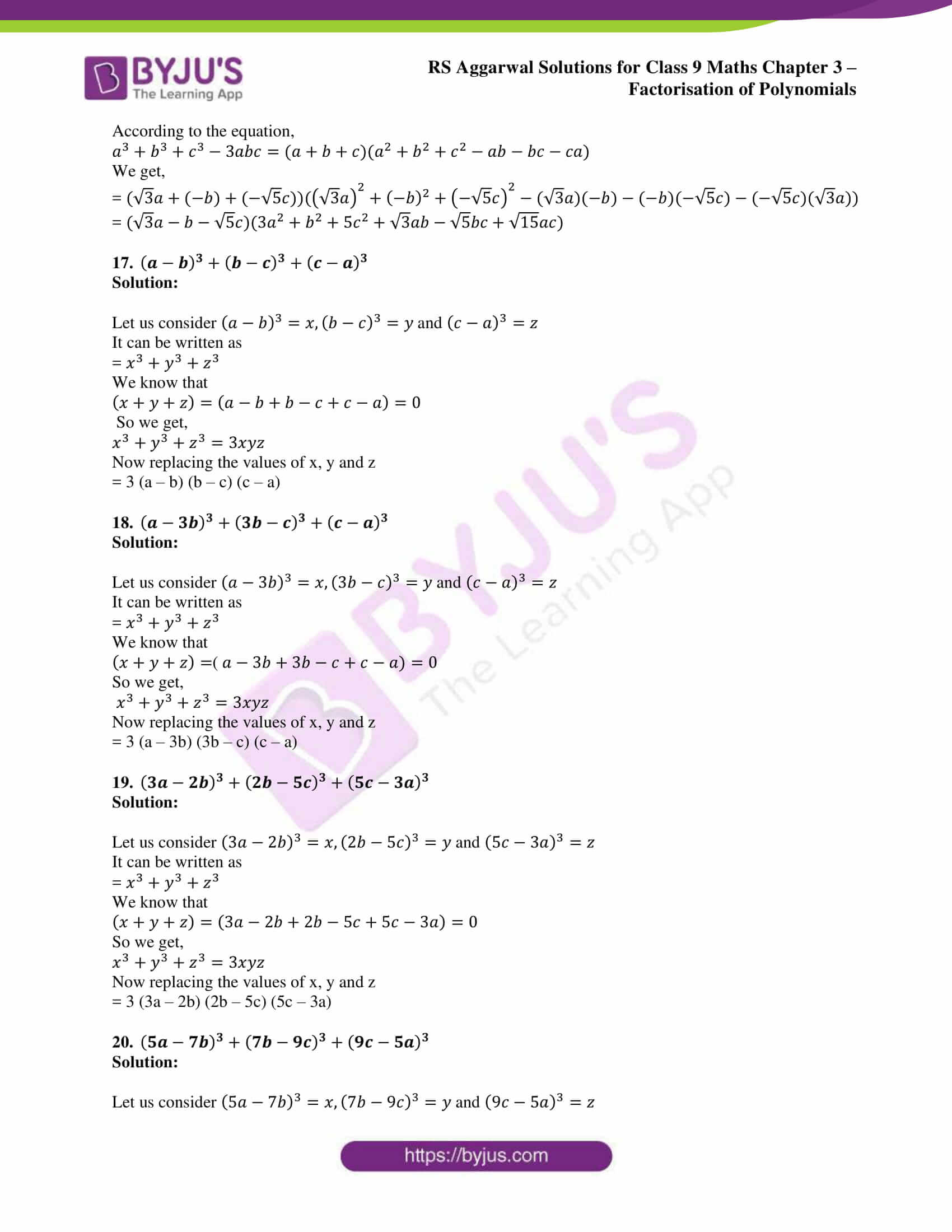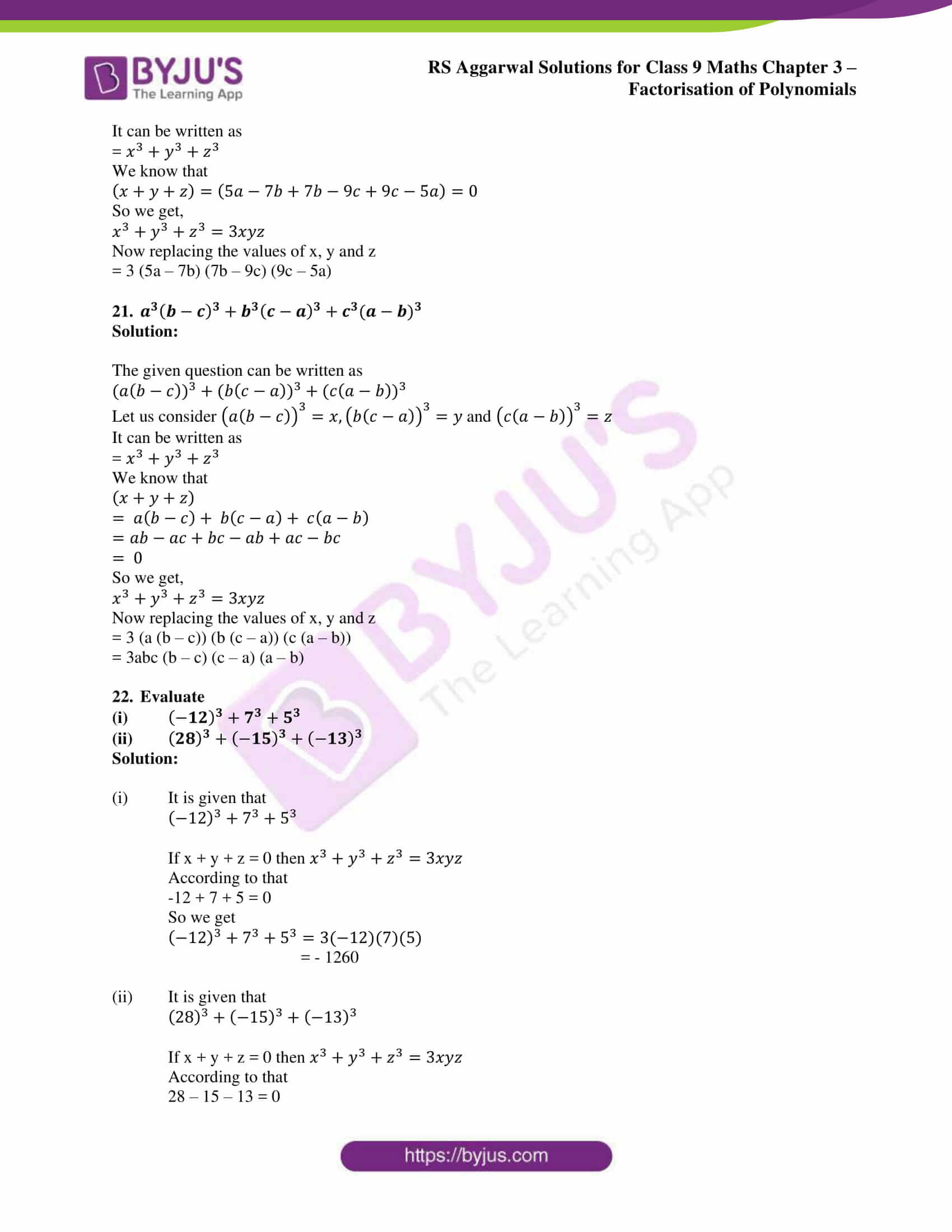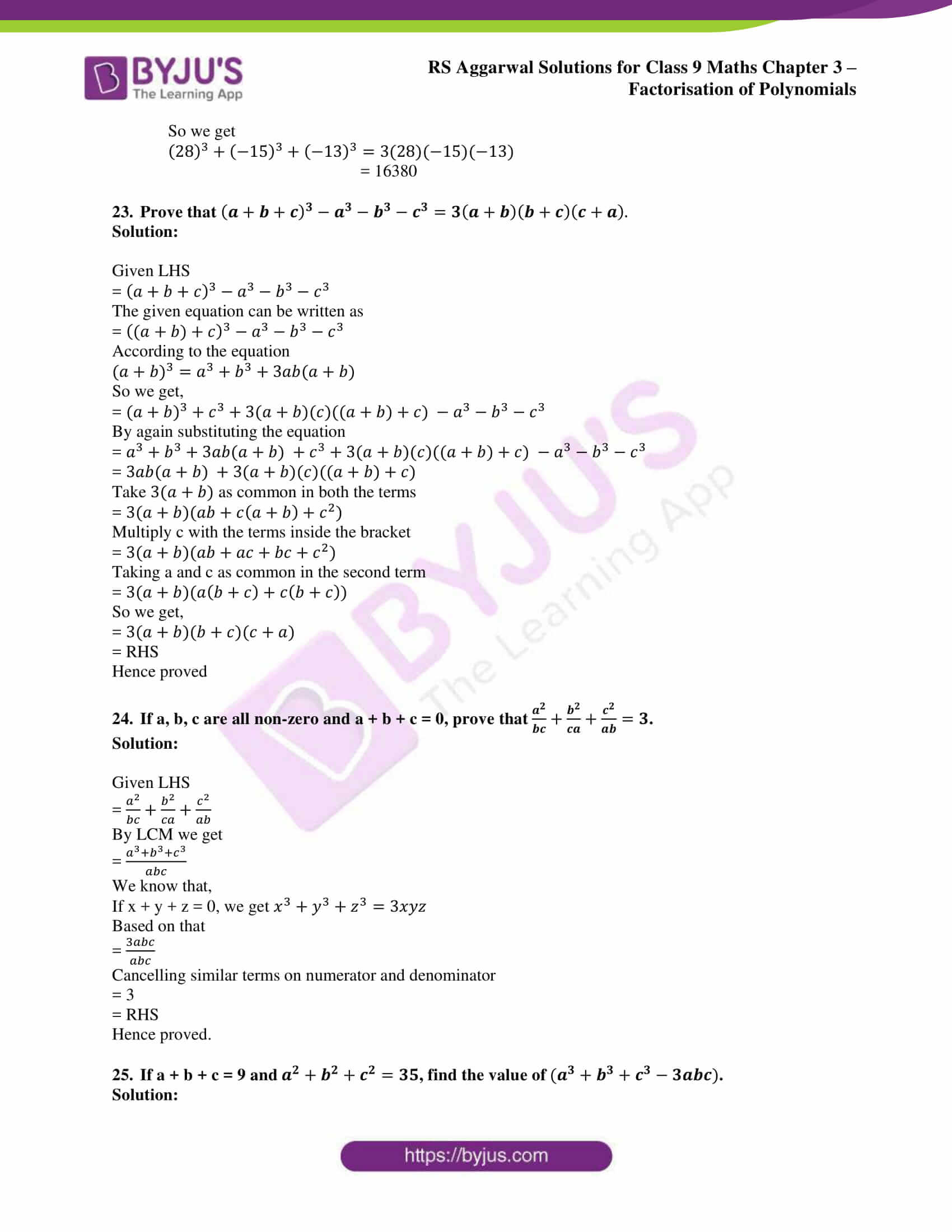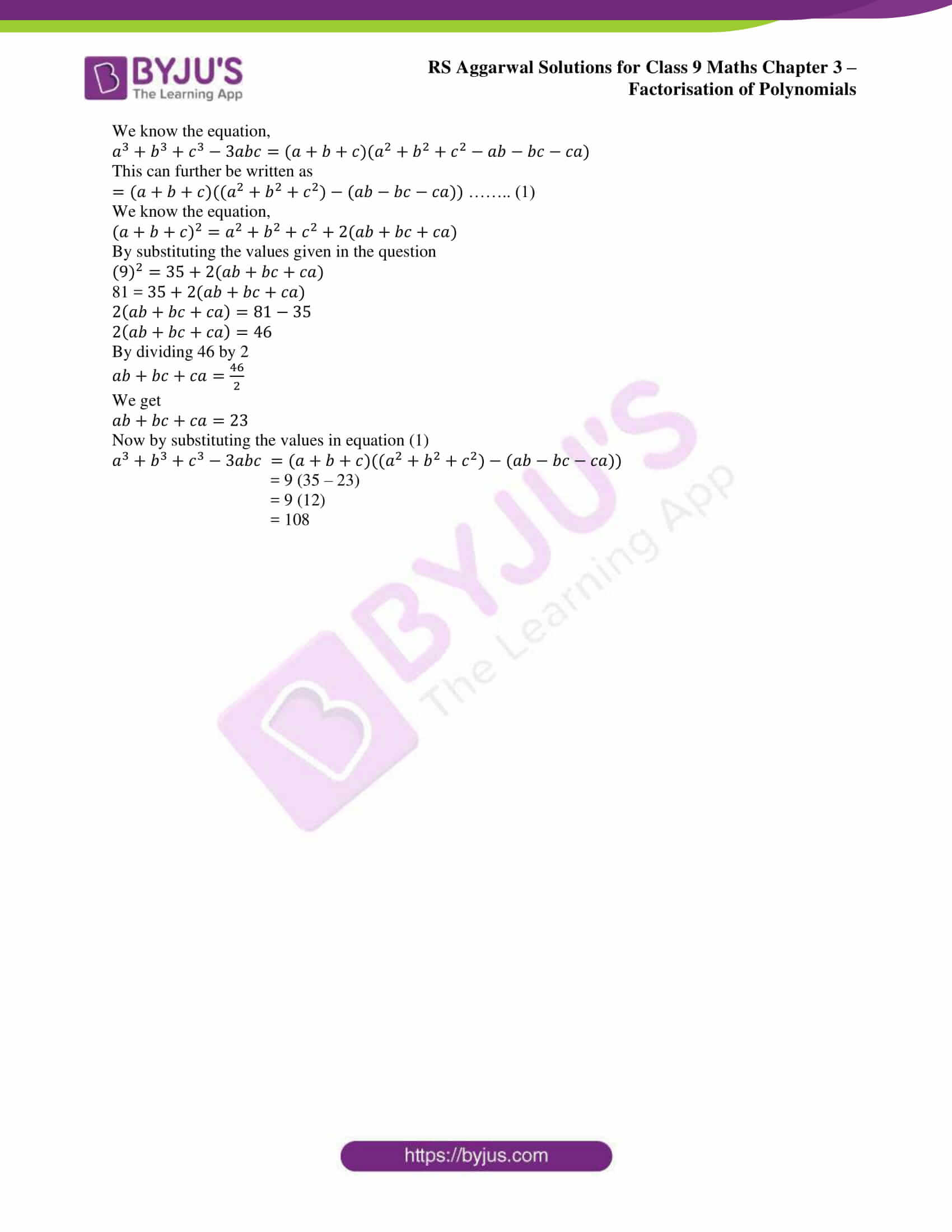### RS Aggarwal Solutions for Class 9 Maths Chapter 3: Factorisation of Polynomials

Chapter 3, Factorisation of Polynomials, has 7 exercises and the RS Aggarwal Solutions has step by step solutions with simple language to help the students understand the topics clearly. Major topics which are covered in this chapter are given below:

• Factor
• Factorisation
• Methods of factorisation
• Factorisation by taking out the common factor
• Factorisation by grouping
• Factorising the difference of two squares